# JP4406428B2 - Signal separation device, signal separation method, signal separation program, and recording medium - Google Patents

Signal separation device, signal separation method, signal separation program, and recording medium Download PDF

## Info

Publication number
JP4406428B2
JP4406428B2 JP2006520556A JP2006520556A JP4406428B2 JP 4406428 B2 JP4406428 B2 JP 4406428B2 JP 2006520556 A JP2006520556 A JP 2006520556A JP 2006520556 A JP2006520556 A JP 2006520556A JP 4406428 B2 JP4406428 B2 JP 4406428B2
Authority
JP
Japan
Prior art keywords
signal
vector
τ
frequency
unit
Prior art date
Legal status (The legal status is an assumption and is not a legal conclusion. Google has not performed a legal analysis and makes no representation as to the accuracy of the status listed.)
Active
Application number
JP2006520556A
Other languages
Japanese (ja)
Other versions
JPWO2006085537A1 (en
Inventor

Original Assignee

Priority date (The priority date is an assumption and is not a legal conclusion. Google has not performed a legal analysis and makes no representation as to the accuracy of the date listed.)
Filing date
Publication date
Priority to JP2005031824 priority Critical
Priority to JP2005031824 priority
Priority to JP2005069768 priority
Priority to JP2005069768 priority
Priority to JP2005166760 priority
Priority to JP2005166760 priority
Priority to PCT/JP2006/302092 priority patent/WO2006085537A1/en
Application filed by 日本電信電話株式会社 filed Critical 日本電信電話株式会社
Publication of JPWO2006085537A1 publication Critical patent/JPWO2006085537A1/en
Application granted granted Critical
Publication of JP4406428B2 publication Critical patent/JP4406428B2/en
Application status is Active legal-status Critical
Anticipated expiration legal-status Critical

## Images

•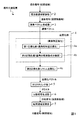•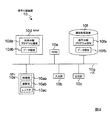•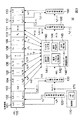•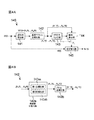•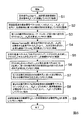•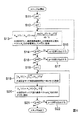••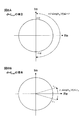•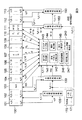•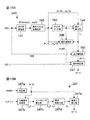•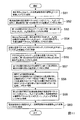•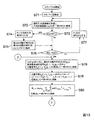•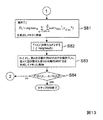•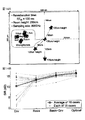•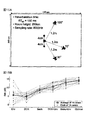•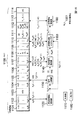•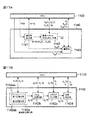•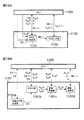•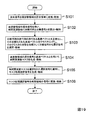•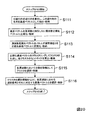•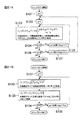•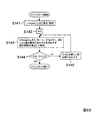•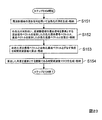•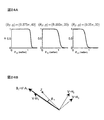•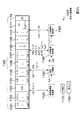•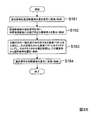•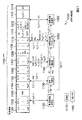•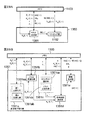•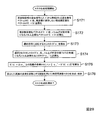••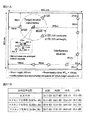•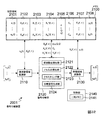•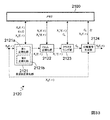•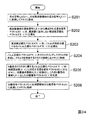•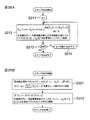••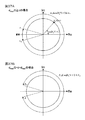•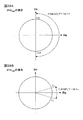•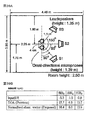•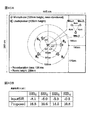•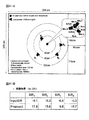## Classifications

• GPHYSICS
• G10MUSICAL INSTRUMENTS; ACOUSTICS
• G10LSPEECH ANALYSIS OR SYNTHESIS; SPEECH RECOGNITION; SPEECH OR VOICE PROCESSING; SPEECH OR AUDIO CODING OR DECODING
• G10L21/00Processing of the speech or voice signal to produce another audible or non-audible signal, e.g. visual or tactile, in order to modify its quality or its intelligibility
• G10L21/02Speech enhancement, e.g. noise reduction or echo cancellation
• G10L21/0272Voice signal separating
• GPHYSICS
• G06COMPUTING; CALCULATING; COUNTING
• G06KRECOGNITION OF DATA; PRESENTATION OF DATA; RECORD CARRIERS; HANDLING RECORD CARRIERS
• G06K9/00Methods or arrangements for reading or recognising printed or written characters or for recognising patterns, e.g. fingerprints
• G06K9/62Methods or arrangements for recognition using electronic means
• G06K9/6217Design or setup of recognition systems and techniques; Extraction of features in feature space; Clustering techniques; Blind source separation
• G06K9/6232Extracting features by transforming the feature space, e.g. multidimensional scaling; Mappings, e.g. subspace methods
• G06K9/624Extracting features by transforming the feature space, e.g. multidimensional scaling; Mappings, e.g. subspace methods based on a separation criterion, e.g. independent component analysis
• G06K9/6244Extracting features by transforming the feature space, e.g. multidimensional scaling; Mappings, e.g. subspace methods based on a separation criterion, e.g. independent component analysis enforcing sparsity or involving a domain transformation
• G06K9/6245Extracting features by transforming the feature space, e.g. multidimensional scaling; Mappings, e.g. subspace methods based on a separation criterion, e.g. independent component analysis enforcing sparsity or involving a domain transformation characterised by a domain transformation
• GPHYSICS
• G10MUSICAL INSTRUMENTS; ACOUSTICS
• G10LSPEECH ANALYSIS OR SYNTHESIS; SPEECH RECOGNITION; SPEECH OR VOICE PROCESSING; SPEECH OR AUDIO CODING OR DECODING
• G10L15/00Speech recognition
• G10L15/20Speech recognition techniques specially adapted for robustness in adverse environments, e.g. in noise, of stress induced speech
• GPHYSICS
• G10MUSICAL INSTRUMENTS; ACOUSTICS
• G10LSPEECH ANALYSIS OR SYNTHESIS; SPEECH RECOGNITION; SPEECH OR VOICE PROCESSING; SPEECH OR AUDIO CODING OR DECODING
• G10L21/00Processing of the speech or voice signal to produce another audible or non-audible signal, e.g. visual or tactile, in order to modify its quality or its intelligibility
• G10L21/02Speech enhancement, e.g. noise reduction or echo cancellation
• G10L21/0208Noise filtering
• G10L21/0216Noise filtering characterised by the method used for estimating noise
• G10L2021/02161Number of inputs available containing the signal or the noise to be suppressed
• G10L2021/02165Two microphones, one receiving mainly the noise signal and the other one mainly the speech signal

## Description

The present invention belongs to the technical field of signal processing, and particularly relates to a technique for extracting a source signal from a mixed signal obtained by mixing a plurality of source signals in a space.

As a conventional technique for extracting a signal by using a plurality of sensors and suppressing other signals, a beamformer (also referred to as beamforming) is widely known (for example, non-patent literature). 1). However, since the beam former requires information on the direction of the target signal, there is a drawback that it is difficult to use in a situation where such information cannot be obtained (or cannot be estimated).
Another newer technique is blind signal separation (BSS: Blind Signal Separation) (see Non-Patent Document 2, for example). This is superior in that it does not require information required by the beamformer, and is expected to be used in various situations. Hereinafter, signal separation by this blind signal separation will be described.

[Blind signal separation]
First, the blind signal separation is formulated. All signals are sampled at a sampling frequency f s and expressed discretely. Further, it is assumed that N signals are mixed and observed by M sensors. The following deals with a situation in which the signal is attenuated / delayed depending on the distance from the signal generation source to the sensor, and the signal may be reflected by a wall or the like to cause distortion of the transmission path. The signal mixed in such a situation is transmitted from the signal source k to the sensor q (q is a sensor number [q = 1,..., M]. K is a signal source number [k = 1, ..., N].) Convolutional mixing with impulse response h qk (r)

Can be expressed as Here, t indicates the sampling time. Further, s k (t) indicates a source signal emitted from the signal source at the sampling time t, and x q (t) indicates a signal observed by the sensor q at the sampling time t. R represents a variable for sweeping.
General impulse response h qk (r) has a pulsed strong response after an appropriate period of time, decays with time. The purpose of blind signal separation is the source signal s 1 (t), ..., s N (t) and impulse response h 11 (r), ..., h 1N (r), ..., h M1 (r), ..., h Without knowing MN (r), only source signals s 1 (t), ..., s N (t) are obtained from the observed signals (hereinafter referred to as "mixed signals") x 1 (t), ..., x M (t) the corresponding separated signals y 1 (t), ..., is to seek y N (t).

[Frequency domain]
Next, a conventional blind signal separation procedure will be described.
Here, the separation operation is performed in the frequency domain. For this purpose, an L-point short-time Fourier transform (STFT) is applied to the mixed signal x q (t) at the sensor q, and a time series for each frequency.

Ask for. Here, f is a frequency and is discretized as f = 0, f s / L,..., F s (L−1) / L (f s is a sampling frequency). Also, τ is a discrete time, and j is an imaginary unit. Furthermore, g (r) is a window function. As a window function, for example, Hanning window

Use a window function with the center of power at g (0). In this case, X q (f, τ) represents the frequency characteristic of the mixed signal x q (t) centered at time t = τ. Since X q (f, τ) includes information over L samples, it is not necessary to obtain X q (f, τ) for every τ, and X q (f , τ).
When processing is performed in the frequency domain, convolutional mixing in the time domain represented by Equation (1) is

And simple mixing at each frequency, and the separation operation is simplified. Here, H qk (f) is a frequency response from the source signal k to the sensor q, and S k (f, τ) is discrete to the source signal s k (t) for a short time according to an equation similar to the equation (2). It has been subjected to Fourier transform. When expression (3) is expressed using a vector,

It becomes. Where X (f, τ) = [X 1 (f, τ),..., X M (f, τ)] T is a mixed signal vector, H k (f) = [H 1k (f) ,. H Mk (f)] T is a vector that summarizes the frequency response from the signal source k to each sensor. [*] T indicates a transposed vector of [*].
[Signal separation by independent component analysis]
One of the blind signal separation methods is signal separation by independent component analysis (ICA). In this ICA method, the separation matrix W (f) and the separation signal vector of N rows and M columns are obtained from only the mixed signal vector X (f, τ).
Y (f, τ) = W (f) X (f, τ) (5)
Is calculated. Here, the separation matrix W (f) is the separation signal vector Y (f, τ) = [Y 1 (f, τ), ..., Y N (f, τ)] T elements (separation signals) Y 1 (f, τ),..., Y N (f, τ) are calculated so as to be independent from each other. There are algorithms described in Non-Patent Document 4 as algorithms for that purpose.

Since the separation is performed by focusing on signal independence in ICA, the obtained separated signals Y 1 (f, τ),..., Y N (f, τ) have an arbitrary order. This is because their independence is maintained even if the order of the signals is changed. The problem of arbitraryness of order is called a permutation problem, and is a very important problem in signal separation in the frequency domain. This permutation problem must be solved so that the subscript p of the separated signal Y p (f, τ) corresponding to the same source signal S k (f, τ) is the same at all frequencies f. I must.
As a conventional method for solving this permutation problem, for example, there is one disclosed in Non-Patent Document 5. In this method, information (direction and distance ratio) related to the position of the signal source is estimated based on the positions of the two selected sensors (sensor pairs). And more detailed position information is obtained by integrating the estimated values of a plurality of sensor pairs. Then, the estimated values as the position information are clustered, and the estimated values belonging to the same cluster are regarded as corresponding to the same signal source to solve the permutation problem.

[Signal separation by time frequency mask]
As another blind signal separation method, there is a method using a time-frequency mask. This method is an effective signal separation and extraction method even when the number N of signal sources and the number M of sensors are in a relationship of M <N.
This method assumes signal sparsity. Sparse refers to the signal being zero at most discrete times τ. The sparsity of the signal is confirmed by an audio signal in the frequency domain, for example. By assuming the sparseness and mutual independence of signals, it can be assumed that even if a plurality of signals exist simultaneously, the probability that they are observed overlapping each other at each time frequency point (f, τ) is low. Thus, mixed signal at each sensor of each time-frequency point (f, tau) may be assumed that time-frequency point (f, tau) in the active one signal s p (f, τ) and consist only. Therefore, the mixed frequency vector M k (f, τ) for clustering the mixed signal vector with an appropriate feature quantity and extracting the mixed signal X (f, τ) corresponding to the time frequency (f, τ) of each cluster C k member. τ),
Y k (f, τ) = M k (f, τ) X Q ' (f, τ)
Thus, each signal is separated and extracted. Here, X Q ′ (f, τ) is one of the mixed signals, and Q′ε {1,..., M}.

As the feature quantity used for clustering, for example, a mixed signal in two sensors (sensor q and reference sensor Q (Q is referred to as a reference value, and a sensor corresponding to the reference value Q is referred to as a reference sensor Q)). Phase difference

Direction of arrival (DOA) of the signal calculated from

(For example, refer nonpatent literature 3). Here, d is the distance between the sensor q and the reference sensor Q, and c is the signal speed. In addition, k-means method (for example, see Non-Patent Document 6) or the like can be used for clustering. Further, as the time frequency mask M k (f, τ), for example, average values θ 1 to , θ 2 to ,..., Θ N to members belonging to the respective clusters C k are obtained,

What was produced | generated as follows can be used. Here, Δ gives a range for extracting a signal. In this method, if Δ is reduced, good separation and extraction performance can be obtained, but nonlinear distortion increases. When Δ is increased, the non-linear distortion is reduced, but the separation performance is deteriorated.
In addition, as a clustering feature amount, the phase difference (formula (8)) of the mixed signals in the two sensors (sensor q and reference sensor Q) and the gain ratio between the two signals

May be used.
BD Van Veen and KM Buckley, "Beamforming: a versatile approach to spacial filtering," IEEE ASSP Magazine, pp. 4-24, April 1988 S. Haykin, eds, "Unsupervised Adaptive Filtering," John-Wiley & Sons, 2000, ISBN 0-471-29412-8 S. Araki, S. Makino, A. Blin, R. Mukai, and H. Sawada, "Underdetermined blind separation for speech in real environments with sparseness and ICA," in Proc. ICASSP 2004, vol. III, May 2004, pp . 881-884 A. Hyvarinen and J. Karhunen and E. Oja, "Independent Component Analysis," John Wiley & Sons, 2001, ISBN 0-471-40540 R. Mukai, H. Sawada, S. Araki and S. Makino, "Frequency Domain Blind Source Separation using Small and Large Spacing Sensor Pairs," in Proc. Of ISCAS 2004, vol. V, pp.1-4, May 2004 . RO Duda, PE Hart, and DG Stork, Pattern Classification, Wiley Interscience, 2nd edition, 2000

However, the above-described conventional technique has a problem in that signal separation processing cannot be performed easily and efficiently using information obtained from observation signals from a plurality of sensors.
For example, in the case of signal separation by independent component analysis, there is a problem that complicated operations are required to accurately solve the permutation problem. That is, in the conventional technique for solving the permutation problem, the direction and the distance ratio are estimated for each sensor pair. Therefore, in order to solve the permutation problem accurately, it is necessary to integrate the estimated values of a plurality of sensor pairs. Further, although this estimated value includes an error, it is necessary to preferentially use a sensor pair that seems to have a smaller error, or to devise an integration method so that the error can be absorbed well. Furthermore, this conventional method has a problem that the position information of the sensor has to be acquired in advance because of the need to estimate the information regarding the position of the signal source. This is disadvantageous when the sensors are arranged irregularly. Further, even if the sensors are regularly arranged, it is difficult to know the arrangement information precisely, and an operation such as calibration is required to solve a more accurate permutation problem.

In the case of the conventional signal separation method using the time-frequency mask, only a method for the case of two sensors has been proposed. Even when there are three or more sensors, two specific sensors q , The feature amount is calculated using only information of Q. This means a reduction in dimension, and the amount of information is lost as compared to the case where all sensors are used. For this reason, the information of all the sensors cannot be used efficiently, and the performance is limited. Further, in order to effectively use the information of all sensors, for example, it is possible to integrate feature amounts obtained by a plurality of sensor pairs in accordance with Non-Patent Document 5, but for this integration, Further processing for feature quantity extraction is necessary, and it seems necessary to devise in integration such as using a sensor pair that seems to have less integration error preferentially. Furthermore, this method also has a problem that it is necessary to know the exact arrangement information of the sensor in advance. This is disadvantageous when the sensors are arranged freely. Further, even if the arrangement is made regularly, it is difficult to know the arrangement information precisely, and an operation such as calibration is required for more accurate signal extraction.

Furthermore, the basic of the blind signal separation process is to separate the mixed signal observed by the sensor and output a plurality of separated signals. However, not all the separated signals are important, and only a part of the separated signals may include a target signal of interest. In such a case, it is necessary to select a separated signal that includes the target signal, but conventional blind signal separation does not provide information on which separated signal includes the target signal. Therefore, it is necessary to determine which separated signal includes the target signal by some other means.

The present invention has been made in view of these points, and provides a technique capable of performing signal separation processing by using information obtained from observation signals from a plurality of sensors easily and efficiently. With the goal.

In the present invention, in order to solve the above-described problem, first, the frequency domain conversion unit converts the mixed signals observed by a plurality of sensors into mixed signals in the frequency domain. Next, a normalization part normalizes the complex vector produced | generated using the mixed signal of the said frequency domain, and produces | generates the normalization vector which excluded the frequency dependence of the said complex vector. Next, the clustering unit clusters the normalized vectors to generate a cluster. Then, signal separation processing is performed using this cluster.
Here, in order to generate the cluster, it is not necessary to directly use the exact position information of each sensor that observes the mixed signal as input information. Each cluster is formed based on information depending on the position of each signal source. Accordingly, in the present invention, signal separation processing can be performed without using strict position information of each sensor.

In the present invention, it is preferable that the normalization unit includes a first normalization unit that normalizes a declination of each element of the complex vector with reference to one specific element included in the complex vector, and a first normalization unit A second normalization unit that divides the declination of each element normalized by the unit by a value proportional to the frequency.
These normalized complex vectors form a cluster depending on the position of the signal source. This makes it possible to perform signal separation processing without using strict position information of each sensor.

In the present invention, it is preferable that the normalization unit further includes a third normalization unit that normalizes a norm of a vector composed of each element normalized by the second normalization unit to a predetermined value.
These normalized complex vectors form a cluster depending on the position of the signal source. Further, by normalizing the norm of the vector composed of the second normalized elements, the clustering process is simplified.
In a preferred first aspect of the present invention, first, the frequency domain converter converts the mixed signals observed by a plurality of sensors into mixed signals in the frequency domain. Next, the separation matrix calculation unit calculates a separation matrix for each frequency using the frequency domain mixed signal, and the inverse matrix calculation unit calculates a generalized inverse matrix of the separation matrix. Next, the basis vector normalization unit normalizes the basis vectors constituting the generalized inverse matrix to calculate a normalized basis vector. Next, the clustering unit clusters the normalized basis vectors to generate a cluster. Thereafter, the permutation calculating unit calculates a permutation for rearranging the elements of the separation matrix using the cluster center vector and the normalized basis vector. Note that the basis vector is a concept included in the complex vector.

In the first aspect of the present invention, the basis vectors are normalized and clustered to calculate a permutation for solving the permutation problem. Therefore, it is not necessary to acquire sensor position information in advance for this clustering. Furthermore, in the present invention, clustering is performed for all elements of the normalized basis vector, and a permutation for solving the permutation problem is calculated from the result. Therefore, an operation for integrating estimation results as in the conventional case is not required.
In the first aspect of the present invention, more preferably, the basis vector normalization unit performs normalization that eliminates the frequency dependence of the basis vector. More preferably, the normalization that eliminates the frequency dependence of the basis vector normalizes the declination of each element of the basis vector with reference to one specific element included in the basis vector, and further each element Is a normalization in which the declination of is divided by a value proportional to the frequency. Such normalization makes it possible to form clusters depending on the position of the signal source.

In the first aspect of the present invention, more preferably, the normalization for eliminating the frequency dependence of the basis vectors is the basis vectors A p (f) (p = 1,..., N, N are the signal sources) For each element A qp (f) (q = 1,..., M, M is the number of sensors observing the mixed signal),

It is normalization which performs the operation of. Where exp is a Napier number, arg [•] is a declination, f is a frequency, j is an imaginary unit, c is a signal transmission speed, Q is a reference value selected from natural numbers less than or equal to M, Let d be a real number. That is, the normalization performed by the calculation of Expression (10) normalizes the declination of each element of the base vector with reference to one specific element included in the base vector, and further converts the declination of each element to the frequency. Normalization by dividing by a value proportional to. This normalization can eliminate the dependence on frequency. Further, the normalization does not require strict sensor placement information.

In addition, the real number d in the above equation (10) is preferably the maximum distance d max between the reference sensor Q corresponding to the element A Qp (f) and another sensor. This is because the accuracy of clustering is generally improved. Details of this will be described later.
Preferably, in the first aspect of the present invention, the basis vector is normalized to a frequency normalized vector that does not depend on the frequency, and the frequency normalized vector is normalized to a normalized basis vector whose norm takes a specified value. The normalized basis vector generated by the above two-step normalization does not depend on the frequency but depends only on the position of the signal source. The clustering process can be simplified by normalizing the norm.

In the first aspect of the present invention, preferably, the permutation is calculated using the envelope of the separated signal (envelope of the absolute value of the separated signal), the center vector of the cluster, and the normalized basis vector. Thereby, the permutation problem can be solved with higher accuracy.
In a preferred second aspect of the invention, the frequency domain conversion unit converts the mixed signal observed by a plurality of sensors into a frequency domain mixed signal, and the signal separation unit uses the frequency domain mixed signal for each frequency. A separation matrix and a separation signal are calculated. Then, the target signal selection unit selects a signal including the target signal from the plurality of separated signals as a selection signal. The procedure is to normalize the basis vectors that are columns of the generalized inverse matrix of the separation matrix, cluster the normalized basis vectors, and determine the selection signal using the variance of the clusters as an index. When the separation matrix is a square matrix, the “generalized inverse matrix” corresponds to an inverse matrix. That is, the generalized inverse matrix here is a concept including a normal inverse matrix.

By using cluster dispersion as an index, a signal close to the sensor can be positioned as a target signal, and a separation signal including such a target signal can be selected as a selection signal. The reason is described below. Here, normalization of the basis vectors is performed when the convolution mixture of signals emitted from a plurality of signal sources is approximated to a predetermined model (for example, a near-field model), and the normalized basis vectors are obtained from the signal source. This is done to form clusters that depend only on the position. However, in the real environment, there are various factors that are not reflected in such a model. For example, in the near field model, transmission distortion caused by reflection of a signal on a wall or the like is not reflected. The difference between the actual environment and the model increases as the distance from the signal source to the sensor increases, and decreases as the signal is closer to the sensor. Therefore, the signal closer to the sensor can be normalized under conditions closer to the real environment, and the cluster variance caused by the difference between the real environment and the model can be reduced. In the second preferred embodiment of the present invention, attention is paid to this relationship, and a selection signal including a target signal that is a signal close to the sensor is extracted using the variance of the cluster as an index. By the above operation, the target signal can be extracted and other interference signals can be suppressed to some extent.

However, when the separation matrix and the separation signal are calculated by independent component analysis (ICA), the number of interference signals that can be completely suppressed by the above processing is up to the number of sensors minus one. That is, when the number of interference signals is more than that, residual components that cannot be suppressed remain. Therefore, preferably in the present invention, the mask generation unit further generates a time frequency mask using the mixed signal and the basis vector in the frequency domain, and the masking unit converts the time frequency mask into the selected selection signal. Apply. Thereby, even when the number of signal sources is larger than the number of sensors, the interference signal remaining in the selection signal can be suppressed better.

Furthermore, in the second aspect of the present invention, preferably, the mask generation unit generates a whitening matrix using the frequency domain mixed signal, and uses the whitening matrix to generate a mixed signal vector having the frequency domain mixed signal as an element. The converted whitened mixed signal vector and the whitened basis vector obtained by converting the basis vector are calculated, and the angle formed by the whitened mixed signal vector and the whitened basis vector is calculated for each time frequency. A time frequency mask is generated using a function to By applying this time frequency mask to the selection signal, the interference signal remaining in the selection signal can be further suppressed.

Still further, in the second aspect of the present invention, it is preferable that the whitening matrix has a frequency f, a discrete time τ, a mixed signal vector X (f, τ), and a vector * which is a time average of a vector ** > τ, and the complex conjugate transposed vector of vector * (vector obtained by transposing the complex conjugate of each element) is set as * H, and R (f) = <X (f, τ) · X (f, τ) H > τ In this case, V (f) = R (f) −1/2 . Then, by calculating Z (f, τ) = V (f) · X (f, τ), the whitened mixed signal vector Z (f, τ) is calculated, and the basis vector is A (f). The whitening basis vector B (f) is calculated by the calculation of B (f) = V (f) · A (f). Also, θ (f, τ) = cos −1 (| B H (f) · Z (f, τ) | when the absolute value of the vector * is | * | and the norm of the vector * is ‖ * ‖. / ‖B (f) ‖ ・ ‖Z (f, τ) ‖) is calculated by calculating the angle θ (f, τ), and α, g, θ T is a real number and the logistic function M (θ ( f, τ)) = α / (1 + eg · (θ (f, τ) −θT) ) is calculated as a time frequency mask. By applying this time frequency mask to the extracted selection signal, the interference signal remaining in the selection signal can be further suppressed.

In the second aspect of the present invention, it is preferable that the target signal selection unit performs normalization that eliminates the frequency dependence of the basis vectors. In the second aspect of the present invention, more preferably, normalization, normalization of the argument of each element of the basis vectors a particular one element comprising the base vectors referenced to eliminate the frequency dependent basis vectors Further, normalization is performed by dividing the declination of each element by a value proportional to the frequency. Furthermore, in the second aspect of the present invention, preferably, the normalization that excludes the frequency dependence of the basis vector is performed by using each element A qp (f) (q = 1) of the basis vector A p (f) (p is a natural number). , ..., M, M is the number of sensors that observe mixed signals)

It is normalization which performs the operation of. Where exp is a Napier number, arg [•] is a declination, f is a frequency, j is an imaginary unit, c is a signal transmission speed, Q is a reference value selected from natural numbers less than or equal to M, Let d be a real number. With such normalization, when the convolution mixture of signals emitted from a plurality of signal sources is approximated to a predetermined model, the normalized basis vectors form a cluster depending on the position of the signal source. . As a result, as described above, it is possible to select a separation signal including a target signal using the size of cluster dispersion as an index. Note that the normalization does not require strict sensor placement information.

Furthermore, it is desirable that the real number d in the above equation (11) is the maximum distance d max between the reference sensor Q and another sensor. This is because the accuracy of clustering is generally improved. Details of this will be described later.
In the second aspect of the present invention, it is preferable that the target signal selection unit selects a cluster having the smallest variance and selects a separation signal corresponding to the selected cluster as the target signal is included. Thereby, the signal with the smallest deviation from the model (for example, the signal closest to the sensor) can be extracted as the target signal.

In a preferred third aspect of the present invention, first, the frequency domain converter converts the mixed signals observed by a plurality of sensors into mixed signals in the frequency domain. Next, a vector normalization part normalizes the mixed signal vector comprised from the mixed signal of a frequency domain, and calculates a normalization vector. Then, the clustering unit clusters the normalized vectors to generate a cluster. Thereafter, the separated signal generation unit extracts a predetermined element from the mixed signal vector corresponding to the time frequency of the normalized vector belonging to the kth cluster, and generates a separated signal vector using this as the kth element.

Here, in the third aspect of the present invention, mixed signals observed by all sensors are normalized to perform clustering, and a separated signal vector is generated using information of each cluster. This means that the separation signal is extracted using the information of all the sensors simultaneously. Also, this process does not require strict sensor placement information. As described above, in the third aspect of the present invention, signal separation can be performed easily and efficiently using information obtained from all the observation signals without requiring strict arrangement information of the sensors.
In the third aspect of the present invention, it is preferable that the vector normalization unit performs normalization that excludes frequency dependence of a mixed signal vector composed of mixed signals in the frequency domain. More preferably, the normalization that eliminates the frequency dependence of the mixed signal vector normalizes the declination of each element of the base vector with reference to one specific element included in the mixed signal vector, and Normalization by dividing the declination of an element by a value proportional to the frequency. More preferably, the normalization for eliminating the frequency dependence of the mixed signal vector is such that each element X q (f, τ) (q = 1,..., M, M of the mixed signal vector observes the mixed signal. Number of sensors)

It is normalization which performs the operation of. Where exp is a Napier number, arg [•] is a declination, j is an imaginary unit, c is a signal transmission speed, Q is a value selected from natural numbers less than or equal to M, d is a real number, and f Is the frequency and τ is the discrete time. As a result, frequency dependence can be eliminated. Thereby, a cluster depending on the position of the signal source can be formed. Note that the normalization does not require strict sensor placement information.

Moreover, it is desirable that the real number d in the above equation (12) is the maximum distance d max between the sensor corresponding to the element X Q (f, τ) and another sensor. This is because the accuracy of clustering is generally improved. Details of this will be described later.
In the third aspect of the present invention, preferably, the vector normalization unit performs normalization that eliminates the frequency dependence of the mixed signal vector and normalization that sets the norm to a specified value. Thereby, the clustering process is simplified.

As described above, in the present invention, signal separation processing can be performed by using information obtained from observation signals from a plurality of sensors easily and efficiently.
For example, in the first invention, the permutation problem can be solved accurately without the need for prior acquisition of information related to strict sensor placement and complicated operations. In the second invention, the target signal can be extracted from the mixed signal obtained by mixing the signals emitted from the plurality of signal sources without knowing the information on the direction of the target signal (even if N> M). Even if). In the third invention, signal separation can be performed easily and efficiently using information obtained from all the observation signals without requiring strict arrangement information of the sensors (even if N> Even if M).

FIG. 1 is a block diagram illustrating the functional configuration of a signal separation device having the principle of the present invention. FIG. 2 is a block diagram illustrating a hardware configuration of the signal separation device according to the first embodiment. FIG. 3 is an example of a block diagram of the signal separation device according to the first embodiment. 4A is a block diagram illustrating details of the permutation problem solving unit in FIG. FIG. 4B is a block diagram illustrating details of the basis vector normalization unit of FIG. 4A. FIG. 5 is a flowchart for explaining the entire processing of the signal separation device according to the first embodiment. FIG. 6 is a flowchart for explaining details of the normalization processing according to the first embodiment. FIG. 7A shows the relationship between the element A qp ″ (f) of the normalized basis vector for each parameter d and its argument arg [A qp ″ (f)] when d max / 2 ≧ d. It is a complex top view for demonstrating. FIG. 7B shows a normalized basis vector element A qp ″ (f) and its argument arg [A qp ″ (f)] for each parameter d when d max / 2 <d <d max . It is a complex plan view for explaining the relationship. FIG. 8A explains the relationship between the element A qp ″ (f) of the normalized basis vector for each parameter d and the argument arg [A qp ″ (f)] when d = d max . FIG. FIG. 8B illustrates the relationship between the element A qp ″ (f) of the normalized basis vector for each parameter d and the argument arg [A qp ″ (f)] when d> d max . FIG. FIG. 9 is an illustration of a block diagram of a signal separation device in the second exemplary embodiment. FIG. 10A is a block diagram illustrating details of the permutation problem solving unit in FIG. FIG. 10B is a block diagram illustrating details of the permutation correction unit in FIG. 10A. FIG. 11 is a flowchart for explaining the entire processing of the signal separation device according to the second embodiment. FIG. 12 is a flowchart for explaining an example of step S58 in FIG. FIG. 13 is a flowchart for explaining an example of step S58 in FIG. FIG. 14A is a diagram showing sound source separation experiment conditions according to the first embodiment and the second embodiment. FIG. 14B is a diagram illustrating a sound source separation experiment result according to the first embodiment and the second embodiment. FIG. 15A is a diagram showing sound source separation experiment conditions according to the first embodiment and the second embodiment. FIG. 15B is a diagram illustrating a sound source separation experiment result according to the first embodiment and the second embodiment. FIG. 16 is a block diagram illustrating the configuration of a signal separation device according to the third embodiment. FIG. 17A is a block diagram illustrating a detailed configuration of the target signal selection unit in FIG. FIG. 17B is a block diagram illustrating a detailed configuration of the basis vector clustering unit in FIG. 17A. FIG. 18A is a block diagram illustrating a detailed configuration of the time-frequency masking unit in FIG. FIG. 18B is a block diagram illustrating details of the mask generation unit in FIG. 18A. FIG. 19 is a flowchart for explaining the entire signal separation processing according to the third embodiment. FIG. 20 is a flowchart for explaining details of the processing of the target signal selection unit in the third embodiment. FIG. 21A is a flowchart for explaining details of the frequency normalization in step S112. FIG. 21B is a flowchart for explaining details of norm normalization in step S113. FIG. 22 is a flowchart illustrating details of the selection signal selection procedure (step S115). FIG. 23 is a flowchart for explaining details of step S104 in FIG. FIG. 24A is a diagram illustrating a time frequency mask M (f, τ) calculated according to the equation (46) for two types of real number parameters θ T , g. FIG. 24B shows a vector corresponding to an interference signal in addition to a vector V (f) · H 1 (f) corresponding to a target signal (I (f) = 1) at a certain time frequency position (f, τ). V (f) · H 2 (f), a diagram V (f) · H 3 to (f) showed that co-exist. FIG. 25 is an illustration of a block diagram of a signal separation device according to the fourth exemplary embodiment. FIG. 26 is a flowchart for explaining processing of the signal separation device according to the fourth embodiment. FIG. 27 is an example of a block diagram of a signal separation device according to the fifth exemplary embodiment. FIG. 28A is a block diagram showing a detailed configuration of the time-frequency masking unit in FIG. FIG. 28B is a block diagram illustrating a detailed configuration of the mask generation unit in FIG. 28A. FIG. 29 is a flowchart for explaining a time-frequency mask generation process according to the fifth embodiment. FIG. 30A is a flowchart for explaining details of step S171 in FIG. FIG. 30B is a flowchart for explaining details of step S172 in FIG. FIG. 31A is a diagram showing experimental conditions for showing the effects of the third embodiment and the fourth embodiment. FIG. 31B is a table showing the average improvement in SIR when only ICA is used (fourth embodiment) and when both ICA and time-frequency masking are combined (third embodiment). FIG. 32 is an example of a block diagram of a signal separation device according to the sixth exemplary embodiment. FIG. 33 is a block diagram illustrating details of the signal separation unit in FIG. FIG. 34 is a flowchart for explaining the overall processing of the signal separation device according to the sixth embodiment. FIG. 35A is a flowchart for explaining details of the processing in step S202 shown in FIG. FIG. 35B is a flowchart for explaining details of the process in step S203 shown in FIG. FIG. 36 is a flowchart for explaining details of the processing in step S205 shown in FIG. FIG. 37A shows an element X q ″ (f, τ) of a norm normalized vector X ″ (f, τ) for each parameter d and its argument arg [X q when d max / 2 ≧ d. '' (F, τ)] is a complex plan view for explaining the relationship. FIG. 37B shows an element X q ″ (f, τ) of a norm normalized vector X ″ (f, τ) for each parameter d and its argument arg in the case of d max / 2 <d <d max. FIG. 6 is a complex plan view for explaining the relationship with [X q ″ (f, τ)]. FIG. 38A shows an element X q ″ (f, τ) of a norm normalized vector X ″ (f, τ) for each parameter d and its argument arg [X q ″ when d = d max . It is a complex plan view for explaining the relationship with (f, τ)]. FIG. 38B shows an element X q ″ (f, τ) of a norm normalized vector X ″ (f, τ) for each parameter d and its argument arg [X q ″ when d> d max . It is a complex plan view for explaining the relationship with (f, τ)]. FIG. 39A is a diagram showing a sound source separation experiment condition according to the sixth embodiment. FIG. 39B is a diagram showing a sound source separation experiment result according to the sixth embodiment. FIG. 40A is a diagram showing a sound source separation experiment condition according to the sixth embodiment. FIG. 40B is a diagram showing a sound source separation experiment result according to the sixth embodiment. FIG. 41A is a diagram showing a sound source separation experiment condition according to the sixth embodiment. FIG. 41B is a diagram showing a sound source separation experiment result according to the sixth embodiment.

Explanation of symbols

1,10,200,1001,1200,1300,2001 Signal separation device

Hereinafter, embodiments of the present invention will be described with reference to the drawings.
〔principle〕
First, the principle of the present invention will be described.
FIG. 1 is a block diagram illustrating a functional configuration of a signal separation device 1 having the principle of the present invention. As will be described later, the signal separation device 1 is configured, for example, by causing a known Neumann computer to execute a predetermined program.
The signal separation device 1 is a device that separates a mixed signal composed of a mixture of source signals emitted from a plurality of signal sources into the source signal. As illustrated in FIG. 1, the signal separation device 1 includes a frequency domain conversion unit 2, a complex vector generation unit 3, a normalization unit 4, and a clustering unit 5. Here, the normalization unit 4 includes a first normalization unit 4a that normalizes the argument of each element of the complex vector with reference to one specific element included in the complex vector, and a first normalization unit 4a. A second normalizing unit 4b that divides the declination of each normalized element by a value proportional to the frequency, and a vector norm that is normalized by the second normalizing unit 4b is normalized to a predetermined value. And a third normalization unit 4c. Note that the frequency dependence of the complex vector is eliminated by the first normalization unit 4a and the second normalization unit 4b (frequency normalization).

When signal separation processing is performed by the signal separation device 1, first, mixed signals (time domain signals) observed by a plurality of sensors are input to the frequency domain conversion unit 2. The frequency domain transform unit 2 transforms mixed signals (time domain signals) observed by a plurality of sensors into frequency domain mixed signals by short-time discrete Fourier transform or the like. Next, the complex vector generation unit 3 generates a complex vector composed of complex elements using the mixed signal in the frequency domain. Next, the normalization unit 4 normalizes the complex vector to generate a normalized vector that excludes the frequency dependence of the complex vector.

In the normalization of the example of FIG. 1, first, the first normalization unit 4a normalizes the declination of each element of the complex vector for each time frequency with reference to one specific element included in the complex vector. To do. As a result, the declination of each element of the complex vector does not depend on the phase or amplitude of the source signal, but only on the relative position and frequency of the signal source with respect to each sensor (details will be described later). Next, the second normalization unit 4b divides the declination of each element normalized by the first normalization unit 4a by a value proportional to the frequency. This eliminates the frequency dependence of each element of each complex vector and normalizes the complex vector to depend only on the relative position of each signal source relative to each sensor. Further, the third normalization unit 4c normalizes the norm of the vector composed of each element normalized by the second normalization unit 4b to a predetermined value.

Next, the clustering unit 5 performs clustering of the normalized vectors that have been subjected to such normalization to generate a cluster. These clusters depend only on the relative position of each signal source with respect to each sensor. The separated signal generation unit 6 performs various signal separation processes using these clusters to generate a frequency domain separated signal. Finally, the time domain conversion unit converts the frequency domain separation signal into a time domain separation signal.
As described above, in order to generate these clusters, it is not necessary to acquire accurate position information of each sensor in advance. In addition, information of observation signals from all sensors is used to generate these clusters. That is, in the present invention, signal separation processing can be performed using information obtained from observation signals from a plurality of sensors easily and efficiently.

Even if the norm is not normalized, it is possible to generate a cluster depending only on the relative position of each signal source with respect to each sensor by devising the clustering method. However, in order to simplify the clustering process, it is desirable to perform norm normalization by the third normalization unit 4c.
Hereinafter, each embodiment of the present invention will be described in detail.
First Embodiment (Example of “First Invention”)]
First, a first embodiment of the present invention will be described.

In this embodiment, the permutation problem is accurately solved without the need for prior acquisition of information related to strict sensor arrangement and complicated operations based on the above-described principle. In this embodiment, a “basis vector” described later corresponds to the “complex vector” described above.
<Hardware configuration>
FIG. 2 is a block diagram illustrating a hardware configuration of the signal separation device 10 according to the first embodiment.
As illustrated in FIG. 2, a signal separation device 10 of this example includes a CPU (Central Processing Unit) 10a, an input unit 10b, an output unit 10c, an auxiliary storage device 10f, a RAM (Random Access Memory) 10d, and a ROM (Read Only). Memory) 10e and bus 10g.

The CPU 10a in this example includes a control unit 10aa, a calculation unit 10ab, and a register 10ac, and executes various calculation processes according to various programs read into the register 10ac. The input unit 10b in this example is an input port for inputting data, a keyboard, a mouse, and the like, and the output unit 10c is an output port for outputting data, a display, and the like. The auxiliary storage device 10f is, for example, a hard disk, an MO (Magneto-Optical disc), a semiconductor memory, or the like, and is observed with a signal separation program area 10fa storing a signal separation program for executing the signal separation processing of this embodiment and a sensor. The data area 10fb stores various data such as mixed signals in the time domain. The RAM 10d is, for example, an SRAM (Static Random Access Memory), a DRAM (Dynamic Random Access Memory) or the like, and has a signal separation program area 10da in which a signal separation program is written and a data area 10db in which various data are written. Yes. The bus 10g in this example connects the CPU 10a, the input unit 10b, the output unit 10c, the auxiliary storage device 10f, the RAM 10d, and the ROM 10e so that they can communicate with each other.

<Cooperation between hardware and software>
The CPU 10a in this example writes the signal separation program stored in the signal separation program area 10fa of the auxiliary storage device 10f to the signal separation program area 10da of the RAM 10d in accordance with the read OS (Operating System) program. Similarly, the CPU 10a writes various data such as a time-domain mixed signal stored in the data area 10fb of the auxiliary storage device 10f to the data area 10db of the RAM 10d. Further, the CPU 10a stores the address on the RAM 10d in which the signal separation program and various data are written in the register 10ac. Then, the control unit 10aa of the CPU 10a sequentially reads these addresses stored in the register 10ac, reads a program and data from the area on the RAM 10d indicated by the read address, and sequentially executes the calculation indicated by the program to the calculation unit 10ab. The calculation result is stored in the register 10ac.

FIG. 3 is an example of a block diagram of the signal separation device 10 configured by reading the signal separation program into the CPU 10a as described above. 4A is a block diagram illustrating details of the permutation problem solving unit 140 in FIG. 3, and FIG. 4B is a block diagram illustrating details of the basis vector normalization unit 142 in FIG. 4A.
As illustrated in FIG. 3, the signal separation device 10 includes a memory 100, a frequency domain conversion unit 120, a separation matrix calculation unit 130, a permutation problem solving unit 140, a separation signal generation unit 150, a time domain conversion unit 160, and a control. Part 170. Further, the permutation problem solving unit 140 of this example includes an inverse matrix calculation unit 141 (corresponding to “complex vector generation unit”), a basis vector normalization unit 142 (corresponding to “normalization unit”), a clustering unit 143, A permutation calculation unit 144 and a rearrangement unit 145 are included, and the basis vector normalization unit 142 includes a frequency normalization unit 142a and a norm normalization unit 142b. Further, the frequency normalization unit 142a includes a first normalization unit 142aa and a second normalization unit 142ab. Further, the control unit 170 has a temporary memory 171.

Here, the memory 100 and the temporary memory 171 correspond to the register 10ac, the data area 10fb of the auxiliary storage device 10f, the data area 10db of the RAM 10d, or the like. In addition, the frequency domain conversion unit 120, the separation matrix calculation unit 130, the permutation problem solving unit 140, the separation signal generation unit 150, the time domain conversion unit 160, and the control unit 170 read the OS program and the signal separation program into the CPU 10a. It is constituted by.
3 and FIG. 4, the broken-line arrows indicate the theoretical information flow, and the solid-line arrows indicate the actual data flow. Also, in these figures, arrows corresponding to the flow of data entering and leaving the control unit 170 are omitted, and arrows corresponding to the actual data flow in FIG. 4 are also omitted.

<Processing>
Next, processing of the signal separation device 10 of this embodiment will be described. In the following, the situation where N source signals are mixed and observed by M sensors will be handled. In the preprocessing, the mixed signal x q (t) (q = 1,..., M) in the time domain observed by each sensor is stored in the storage area 101 of the memory 100, and the signal transmission speed c, It is assumed that a reference value Q selected from natural numbers equal to or less than M (subscript number of one reference sensor selected from M sensors) and each parameter of a real number d are stored in the storage area 107.

FIG. 5 is a flowchart for explaining the entire processing of the signal separation device 10 according to the first embodiment. Hereinafter, the processing of the signal separation device 10 according to this embodiment will be described with reference to FIG.
[Processing of Frequency Domain Transformer 120]
First, the frequency domain transform unit 120 reads the time domain mixed signal x q (t) from the storage area 101 of the memory 100, and performs a time series signal (“frequency domain mixing signal "is referred to as a) X q (f, τ) (q = 1, ..., is converted to M), it is stored in the storage area 102 in the memory 100 (step S1).

[Processing of Separation Matrix Calculation Unit 130]
Next, the separation matrix calculation unit 130 reads the frequency domain mixed signal X q (f, τ) from the storage area 102 of the memory 100. Mixed signals X q in the frequency domain (f, tau) separating matrix calculation unit 130 reads the mixed signal vector X (f, τ) consisting = [X 1 (f, τ ), ..., X M (F, τ)] T , and by independent component analysis (ICA), the first separation matrix W (f) and the separation signal vector Y (f, τ) = [Y 1 (f, τ), ..., Y N (f, τ)] T is calculated. The calculated first separation matrix W (f) is stored in the storage area 103 of the memory 100 (step S2).

Here, the first separation matrix W (f) calculated by the separation matrix calculation unit 130 includes arbitrary order. Therefore, in the permutation problem solving unit 140 as described below, the first separation matrix W (f) is changed to the second separation matrix W ′ (f) in which the arbitrary order is solved.
[Process of Permutation Problem Solving Unit 140]
First, the inverse matrix calculation unit 141 reads the first separation matrix W (f) from the storage area 103 of the memory 100, and the Moore-Penrose generalized inverse matrix W + (f) = [A 1 (f), ..., A N (f)] (when M = N, it matches the inverse matrix W -1 (f)), and the basis vector A p (f) = [ A 1p (f),..., A Mp (f)] T (p = 1,..., N) is stored in the storage area 104 of the memory 100 (step S3) .

Next, in the basis vector normalization unit 142, the basis vector A p (f) (p = 1,..., N, f = 0, f s / L,..., F s ( L-1) / L) is read out, and a normalized basis vector A p ″ (f) obtained by normalizing these is calculated and stored in the storage area 106 of the memory 100 (step S4). Note that the basis vector normalization unit 142 generates all basis vectors A p (f) (p = 1,..., N, f = 0, f s / L,..., F s (L−1) / L ) And normalize them to a normalized basis vector A p ″ (f) that does not depend on frequency but depends only on the position of the signal source. As a result, when they are clustered, each cluster corresponds to each signal source. If this normalization is not performed properly, clusters will not be formed. Also, normalization in this embodiment consists of two stages, frequency normalization and norm normalization. In the frequency normalization, the frequency normalization unit 142a (FIG. 4B) normalizes the base vector to a frequency normalization vector independent of frequency. In norm normalization, the norm normalization unit 142b normalizes the frequency normalized vector to a normalized basis vector whose norm takes a specified value (1 in this example). Details of these normalization processes will be described later.

Then, the clustering section 143, 'reads the (f), these normalized basis vectors A p' from the storage area 106 of the memory 100 normalized basis vectors A p 'of N by clustering' (f) cluster C k (k = 1,..., N) is generated, and information specifying each cluster C k and their centroid (center vector) η k is stored in the storage areas 108 and 109 of the memory 100 (steps). S5). This clustering is performed by, for example, the sum U of square sums U k between the elements of each cluster C k (normalized basis vectors A v ″ (f)) and the centroid η k of each cluster C k.

Is performed on the basis of minimizing. This minimization can be effectively performed by using k-means clustering described in Non-Patent Document 6, for example. It should be noted that the centroid η k of cluster C k is,

Calculated by Here, | C k | is the number of elements (normalized basis vectors A v ″ (f)) in the cluster C k . Here, the square of the Euclidean distance is used as the distance, but a Minkowski distance or the like generalized from this may be used. The reason why the normalized basis vector A p ″ (f) forms a cluster will be described later.
Next, the permutation calculation unit 144 reads the normalized basis vector A p ″ (f) from the storage area 106 of the memory 100 and the centroid η k of each cluster C k from the storage area 109. Then, the permutation calculation unit 144 uses these to reorder the elements of the first separation matrix W (f) from the permutations ｆ f ({1,2, ..., N} to {1,2, ..., N). } Is calculated for each frequency f and stored in the storage area 110 of the memory 100 (step S6). Note that this permutation はf is

Determined by. Here, argmin Π · in Equation (13) means Π that minimizes . As a procedure for determining the permutation Π f according to the equation (13), for example, for all possible permutations Π (N! Streets)

And a procedure for determining Π corresponding to the minimum value as the permutation ｆ f . A specific example is shown below.
[Specific example 1 of determining permutation Π f ]
The number N of signal sources is 3, normalized basis vectors A 1 ″ (f), A 2 ″ (f), A 3 ″ (f) at a certain frequency f, and the centroids η 1 , η 2. , Η 3 is the square of the distance as shown in the table below.

In this case, the permutation obtained by equation (13) is
Π f : [1,2,3] → [2,3,1]
It becomes. Because
‖Η 1 -A π (1) '' (f) ‖ 2 = ‖η 1 -A 2 '' (f) ‖ 2 = 0.1
‖Η 2 -A π (2) '' (f) ‖ 2 = ‖η 2 -A 3 '' (f) ‖ 2 = 0.2
‖Η 3 -A π (3) '' (f) ‖ 2 = ‖η 3 -A 1 '' (f) ‖ 2 = 0.15
The combination of

(The end of the description of the specific example 1 for determining the permutation ｆ f ).
However, this procedure is not practical as N increases. Therefore, as its approximation, sequentially ‖η k -A Π (k) ' ' (f) ‖ 2 A [pi to the minimum value of the (k) '' (f) will be chosen to no overlap of the Alternatively, a procedure such as a permutation をf for transferring the selected A Π (k) '' (f) to the normalized basis vector A k '' (f) may be used. A procedure for determining the permutation ｆ f by applying this approximation method to the same conditions as those in [Specific example 1 of determining permutation ｆ f ] will be described below.

[Specific example 2 of determining permutation Π f ]
First, in the case of Table 1 above, since the minimum value of the square of the distance is 0.1 (the square of the distance between the normalized basis vector A 2 ″ (f) and each centroid η 1 ), Π (1 ) = 2. Then, erasing the row and column associated with the normalized basis vector A 2 ″ (f) and each centroid η 1 is as follows.

In the case of Table 2, since the minimum value of the square of the distance is 0.15 (the square of the distance between the normalized basis vector A 1 ″ (f) and each centroid η 3 ), Π (3) = 1 To decide. Finally, the remaining 3 is assigned to Π (2) (end of description of [specific example 2 of permutation Π f determination]).
Next, the rearrangement unit 145 reads the first separation matrix W (f) from the storage area 103 of the memory 100 and the permutation Π f from the storage area 110. The rearranging unit 145 stores the row of the first separation matrix W (f) to generate a second separation matrix W '(f) rearranged in accordance with the permutation [pi f in the storage area 111 of the memory 100 (Step S7). Note that the rearrangement of the rows of the first separation matrix W (f) according to the permutation f means that the element A Π (k) '' (f) in the Moore-Penrose generalized inverse matrix W + (f) described above This means that the sorting corresponding to the sorting from A k ″ (f) to A k ″ (f) is performed on the first separation matrix W (f). That is, the rearrangement is performed so that the permutation Π f (k) row of the first separation matrix W (f) becomes the k row of the second separation matrix W ′ (f). In the above-described [specific examples 1 and 2 of determining the permutation ｆ f ], the second, third, and first rows of the first separation matrix W (f) are the first and second separation matrices W ′ (f), respectively. Second and third lines.

[Processing of Separated Signal Generation Unit 150]
Thereafter, the separated signal generation unit 150 reads the mixed signal X q (f, τ) in the frequency domain from the storage area 102 of the memory 100 and the second separation matrix W ′ (f) from the storage area 111. Then, the separated signal generating section 150, the mixed signal in the frequency domain X q (f, τ) consisting of mixed signal vector X (f, τ) = [ X 1 (f, τ), ..., X M (f , tau)] using the T and the second separation matrix W '(f), the separated signal vector
Y (f, τ) = W '(f) · X (f, τ)
, And a frequency domain signal (referred to as a “frequency domain mixed signal”) Y p (f, τ), which is each of these elements, is stored in the storage area 112 of the memory 100 (step S8).

[Processing of Time Domain Conversion Unit 160]
Finally, in the time domain conversion unit 160, the frequency domain separation signal Y p (f, τ) is read from the storage area 112 of the memory 100, and this is shortened for each subscript p (for each Y p (f, τ)). The time domain separation signal y p (t) is converted by time inverse Fourier transform or the like, and the time domain separation signal y p (t) is stored in the storage area 113 of the memory 100 (step S9).
[Details of Normalization Processing (Details of Step S4)]
Next, details of the normalization processing in the above-described basis vector normalization unit 142 (details of step S4) will be described.

FIG. 6 is a flowchart for explaining the details of the normalization process.
First, 1 is substituted for the parameter p in the control unit 170 (FIG. 3), and this is stored in the temporary memory 171 (step S11). In addition, the control unit 170 substitutes 1 for the parameter q and stores it in the temporary memory 171 (step S12). Next, in the frequency normalization unit 142a (FIG. 4), the aforementioned parameters d, c and Q are read from the storage area 107 of the memory 100, the parameters p and q are read from the temporary memory 171 and the basis vector A p (f). For element A qp (f) of

The calculation result A qp ′ (f) is stored in the storage area 105 of the memory 100 as each element A qp ′ (f) of the frequency normalized vector A p ′ (f) (step S13). Note that arg [•] means a declination, and j means an imaginary unit.
More specifically, first, the first normalizing section 142aa of the frequency normalizing section 142a is, basis vector A p (f) of a particular one element A Qp (f) the basis vectors based on the A p (f) The normal angle of each element A qp (f) is normalized by the following calculation.

Next, the second normalization unit 142ab of the frequency normalization unit 142a converts the declination of each element A qp '''(f) normalized by the first normalization unit 142aa to the frequency f as follows. Divide by the proportional value 4fc -1 d.

Next, the control unit 170 determines whether or not the parameter q stored in the temporary memory 171 satisfies q = M (step S14). If not q = M, the control unit 170 sets the calculation result of q + 1 as a new parameter q value, stores it in the temporary memory 171 (step S15), and returns the process to step S13. On the other hand, if q = M, the controller 170 further determines whether or not p = N is satisfied (step S16).

If p = N is not satisfied, the controller 170 sets the calculation result of p + 1 as a new parameter p value, stores it in the temporary memory 171 (step S17), and returns the process to step S12. On the other hand, if p = N, the control unit 170 assigns 1 to the parameter p, stores it in the temporary memory 171 (step S18), and starts processing in the norm normalization unit 142b. First, in the norm normalization unit 142b, each element A qp ′ (f) of the frequency normalized vector A p ′ (f) is read from the storage area 105 of the memory 100,

Performing operations, 'norm ‖A p of (f)' (f) ‖ look frequency normalized vector A p, the frequency normalized vector A p '(f) and its norm ‖A p' (f) || Is stored in the temporary memory 171 (step S19).
Next, the norm normalization unit 142b reads the frequency normalization vector A p ′ (f) and its norm ‖A p ′ (f) ‖ from the temporary memory 171;
A p ″ (f) = A p ′ (f) / ‖A p ′ (f) ‖ (18)
The normalized basis vector A p ″ (f) is obtained by performing the above operation, and is stored in the storage area 106 of the memory 100 (step S20).

Thereafter, the control unit 170 determines whether or not the parameter p stored in the temporary memory 171 satisfies p = N (step S21). If p = N is not satisfied, the control unit 170 sets the calculation result of p + 1 as a new parameter p value, stores it in the temporary memory 171 (step S22), and returns to the process of step S19. On the other hand, if p = N, the controller 170 ends the process of step S4.
The normalized basis vector A p ″ (f) generated in this way is a vector that does not depend on the frequency but depends only on the position of the signal source. As a result, this normalized basis vector A p ″ (f) forms a cluster. The reason for this will be described below.

[Reason why normalized basis vector A p ″ (f) forms a cluster]
Each element A qp (f) of the basis vector A p (f) is proportional to the frequency response H qk from the signal source k to the sensor q corresponding to the source signal p (a complex scalar is applied). Yes. These complex scalars change according to the discrete time, but the relative values of the complex scalars corresponding to the source signal p and the sensor q and the complex scalars corresponding to the source signal p and the sensor Q change in discrete time. Even if it is constant (if the frequency f is the same). That is, if the frequency f is the same, the relative value of the argument of the complex scalar corresponding to the source signal p and the sensor q and the argument of the complex scalar corresponding to the source signal p and the sensor Q are constant. .

As described above, the first normalizing section 142aa of the frequency normalizing section 142a, each of the basis vectors A p (f) a specific one element A Qp basis vectors A p (f) (f) is based Normalizes the declination of element A qp (f). As a result, the uncertainty due to the phase of the complex scalar described above is removed, and the deviation angle of the element A qp (f) of the basis vector A p (f) corresponding to the source signal p and the sensor q is changed to the source signal p. And the reference sensor Q (corresponding to the reference value Q) are expressed as relative values with respect to the deflection angle of the element A Qp (f) of the basis vector A p (f). In this case, the relative value corresponding to the argument of the element A Qp (f) is expressed as 0. The frequency response from the signal source k to the sensor q is approximated by a direct wave model without reflection or reverberation. Then, the deviation angle normalized by the first normalization unit 142aa is proportional to both the arrival time difference of the wave from the signal source k to the sensor and the frequency f. Here, the arrival time difference is the time difference between the time until the wave from the signal source k reaches the sensor q and the time until the wave reaches the reference sensor Q.

Further, as described above, the second normalization unit 142ab divides the declination of each element A qp ″ (f) normalized by the first normalization unit 142aa by a value proportional to the frequency f. As a result, each element A qp ′ ″ (f) is normalized to each element A qp ′ (f) excluding the frequency dependence of the declination angle. Thereby, each normalized element A qp ′ (f) depends only on the arrival time difference of the wave from the signal source k to the sensor according to the direct wave model. Here, the difference in arrival time of waves from the signal source k to the sensor depends only on the relative positions of the signal source k, the sensor q, and the reference sensor Q. Therefore, if the signal source k, the sensor q, and the reference sensor Q are the same, the declination angle of each element A qp ′ (f) is the same even if the frequency f is different. Therefore, the frequency normalization vector A p ′ (f) does not depend on the frequency f, but only on the position of the signal source k.

Therefore, a cluster corresponding to the same signal source is formed by clustering of the normalized basis vector A p ″ (f) obtained by normalizing the norm of the frequency normalized vector A p ′ (f). In an actual environment, the direct wave model is not exactly satisfied due to the influence of reflection, reverberation, etc., but it is a sufficiently good approximation as shown in the experimental results described later.
Next, the reason why the normalized basis vector A p ″ (f) forms a cluster will be described using a model. Approximating the impulse response h qk (r) shown in the above equation (1) using a direct wave (near field) mixing model and expressing it in the frequency domain

It becomes. Here, d qk is the distance between the signal source k and the sensor q, the attenuation 1 / d qk is determined by the distance d qk , and the delay (d qk -d Qk ) / c is the reference sensor Q It is determined by the distance normalized at the position of.
In addition, considering the arbitrary order and the arbitrary scaling in independent component analysis (ICA), the vector that summarizes the frequency response from the signal source k to each sensor in the basis vector A p (f) and Equation (4). The following relationship holds with H k (f).

A p (f) = ε p · H k (f), A qp (f) = ε p · H qk (f) (20)
Here, ε p is a complex scalar value expressing the arbitraryness of scaling, and the possibility that the subscripts k and p are different expresses the arbitraryness of the order. Here, from the equations (16), (18), (19), and (20),

It becomes. As can be seen from this equation, each element A qp ″ (f) of the normalized basis vector A p ″ (f) is independent of the frequency f and depends only on the positions of the signal source k and the sensor q. . Therefore, when the normalized basis vectors A p ″ (f) are clustered, corresponding clusters are formed for the same signal sources.
The same can be said for the case of modeling with a near-field mixed model that does not consider signal attenuation. That is, when the convolutional mixing model shown in the above equation (1) is approximated by a near-field mixing model that ignores attenuation, and expressed in the frequency domain,

It becomes. In this case, from the equations (16), (18), (20), and (22),

It becomes. Also in this case, each element A qp ″ (f) of the normalized basis vector A p ″ (f) is independent of the frequency f and depends only on the positions of the signal source k and the sensor q.
Furthermore, the same can be said for not only the near-field mixed model but also the far-field mixed model. In other words, when the convolutional mixing model shown in the above equation (1) is approximated by a far-field mixing model and expressed in the frequency domain,

It becomes. SE q and SE Q are vectors indicating the positions of the sensors q and Q. Θ k qQ represents an angle formed by a straight line connecting the sensors q and Q and a straight line connecting the center point of the sensors q and Q and the signal source k. In this case, from the equations (16), (18), (20), and (24),

It becomes. Also in this case, each element A qp ″ (f) of the normalized basis vector A p ″ (f) is independent of the frequency f and depends only on the positions of the signal source k and the sensor q.
Further, the value of the parameter d from equation (21), d> is preferably a d max / 2 (d max mean the maximum distance between the reference sensor Q and other sensors that correspond to elements A Qp (f) It is understood that d ≧ d max is more preferable, and d = d max is even more preferable. Hereinafter, this reason will be described.

FIG. 7 and FIG. 8 are complex plan views for explaining the relationship between the element A qp ″ (f) of the normalized basis vector for each parameter d and the argument arg [A qp ″ (f)]. is there. In these figures, the horizontal axis represents the real axis and the vertical axis represents the imaginary axis.
FIG. 7A is a complex plan view when d max / 2 ≧ d. Here, from the above definition of d max , the absolute value of d qk -d Qk is less than or equal to d max for any q and k. Therefore, when d max / 2 ≧ d, (π / 2) · (d qk −d Qk ) / d ≦ −π and (π / 2) · (d qk −d Qk ) / d ≧ π can be obtained. As a result, equation (21) A qp 'represented by' deflection angle (f) arg [A qp ' ' (f)] is greater than 2π α 1 ≦ arg [A qp '' (f)] ≦ There is a possibility of distribution in the range of α 21 ≦ −π, α 2 ≧ π). Therefore, there is a possibility that the declination angles of elements A qp ″ (f) of different normalized basis vectors match. In the clustering described above, different normalized basis vectors A p ″ (f) are clustered into the same cluster. There is a possibility that. Therefore, it is desirable that d> d max / 2. However, if there is no sample of the normalized basis vector A p ″ (f) corresponding to this overlapping range of declination, there is no problem even if d max / 2 ≧ d.

FIG. 7B is a complex plan view in the case of d max / 2 <d <d max . In this case, −π <(π / 2) · (d qk −d Qk ) / d <−π / 2, π / 2 <(π / 2) · (d qk −d Qk ) / d <π obtain. As a result, equation (21) A qp 'represented by' deflection angle (f) arg [A qp ' ' (f)] is, β 1 ≦ arg [A qp '' (f)] ≦ β 2 ( There is a possibility of distribution in the range of −π <β 1 <−π / 2, π / 2 <β 2 <π). Therefore, in the range of −π <arg [A qp ″ (f)] <− π / 2 and π / 2 <arg [A qp ″ (f)] <π, the deviation between different frequency normalized vector elements is As the angle difference increases, the distance between these frequency normalized vector elements may not increase monotonically. This may reduce the accuracy of the clustering described above. Therefore, it is more desirable that d ≧ d max .

FIG. 8A is a complex plan view in the case of d = d max , and FIG. 8B is a complex plan view in the case of d> d max . Here, when d> d max , −π / 2 <(π / 2) · (d qk −d Qk ) / d <0, 0 <(π / 2) · (d qk −d Qk ) / d < It can be π / 2. As a result, equation (21) A qp 'represented by' deflection angle (f) arg [A qp ' ' (f)] , as shown in FIG. 8B, γ 1 ≦ arg [A qp '' ( f)] ≦ γ 2 (−π / 2 <γ 1 <0, 0 <γ 2 <π / 2). As d increases, the distribution range becomes narrower, and clusters are concentrated in a narrow range. This reduces the accuracy of the clustering described above.

On the other hand, when d = d max , −π / 2 ≦ (π / 2) · (d qk −d Qk ) / d <0, 0 <(π / 2) · (d qk −d Qk ) / d ≦ π / 2. As a result, equation (21) A qp 'represented by' deflection angle (f) arg [A qp ' ' (f)] is - [pi] / 2 ≦ arg as shown in Figure 8A [A qp '' ( f)] is distributed in the range of ≦ π / 2. In this case, it is possible to disperse the clusters over as wide a range as possible while maintaining the relationship that the distance increases monotonously with respect to the increase in the difference in declination between the elements of the frequency normalization vector. As a result, generally the accuracy of clustering can be improved.
[Second Embodiment (Example of "First Invention")]
Next, a second embodiment of the present invention will be described.

In the first embodiment, the permutation problem is solved by the information obtained from the basis vectors. However, in this embodiment, Japanese Patent Application Laid-Open No. 2004-145172 and “H. Sawada, R. Mukai, S. Araki” , S. Makino, "A Robust and Precise Method for Solving the Permutation Problem of Frequency-Domain Blind Source Separation," IEEE Trans. Speech and Audio processing, vol. 12, no. 5, pp. 530-538, Sep. 2004 (Hereinafter referred to as “references”) ”, the permutation problem can be solved more accurately by integrating the envelope information of the separated signal. In these documents, information on the direction of the signal source is used instead of the basis vector.

Below, it demonstrates centering around difference with 1st Embodiment, and abbreviate | omits description about the matter which is common to this.
<Configuration>
FIG. 9 is an example of a block diagram of a signal separation device 200 according to the second embodiment. Note that the signal separation device 200 of this embodiment is also configured by reading a signal separation program into the CPU 10a (FIG. 2). 10A is a block diagram illustrating details of the permutation problem solving unit 240 in FIG. 9, and FIG. 10B is a block diagram illustrating details of the permutation correcting unit 247 in FIG. 10A. In FIG. 9 and FIG. 10, the same reference numerals as those in the first embodiment are assigned to portions common to the first embodiment. 9 and 10 indicate the theoretical information flow, and the solid arrow indicates the actual data flow. Further, in these figures, arrows corresponding to the data flow entering and exiting the control unit 170 are omitted, and arrows corresponding to the actual data flow in FIG. 10 are also omitted.

The difference of the present embodiment from the first embodiment is mainly the configuration of the permutation problem solving unit 240. That is, the permutation problem solving unit 240 of this embodiment is obtained by adding a permutation evaluation unit 246 and a permutation correcting unit 247 to the permutation problem solving unit 140 of the first embodiment (see FIG. 9, FIG. 10A). The permutation evaluation unit 246 evaluates the reliability of the permutation for each frequency, and the permutation correction unit 247 uses the envelope of the separation signal for a frequency evaluated as having a low permutation reliability. A simple permutation is calculated. The permutation correction unit 247 includes a determination unit 247a, a separated signal generation unit 247b, an envelope calculation unit 247c, a permutation recalculation unit 247d, and a rearrangement unit 247e (FIG. 10B). In this embodiment, the permutation calculation unit 144 and the permutation correction unit 247 constitute a “permutation calculation unit” in claim 4.

<Processing>
FIG. 11 is a flowchart for explaining the entire processing of the signal separation device 200 according to the second embodiment. Hereinafter, the processing of the signal separation device 200 according to this embodiment will be described with reference to FIG.
Since the process of step S51 to S57 is the same as that of step S1 to S7 in 1st Embodiment, description is abbreviate | omitted. In this embodiment, after the processing of step S57, the permutation evaluation unit 246 evaluates the reliability of the permutation Π f for each frequency, and the separation signal of the permutation Π f is evaluated for the frequency evaluated as having low reliability. Calculate the permutation Π f ′ using the envelope, and rearrange the rows of the second separation matrix W ′ (f) for this frequency only according to the permutation Π f ′ to generate the third separation matrix W ″ (f) Then, it is stored in the storage area 110 of the memory 100 (step S58). Details of this process will be described later.

Thereafter, the separation signal generation unit 150 reads the mixed signal X q (f, τ) in the frequency domain from the storage area 102 of the memory 100 and the third separation matrix W ″ (f) from the storage area 111. Then, the separated signal generating section 150, the mixed signal in the frequency domain X q (f, τ) consisting of mixed signal vector X (f, τ) = [ X 1 (f, τ), ..., X M (f , τ)] T and the third separation matrix W ″ (f), the separation signal vector
Y (f, τ) = W '' (f) ・ X (f, τ)
And the frequency domain separation signal Y p (f, τ) is stored in the storage area 112 of the memory 100 (step S59).

Finally, the time domain conversion unit 160 reads the frequency domain separation signal Y p (f, τ) from the storage area 112 of the memory 100 and converts it into the time domain separation signal y p (t) for each subscript p. The time domain separation signal y p (t) is stored in the storage area 113 of the memory 100 (step S60).
[Details of processing in step S58]
12 and 13 are flowcharts for explaining an example of step S58 in FIG. Details of step S58 will be described below along this flowchart.

First, the control unit 170 substitutes 0 for the parameter f, sets the set F as an empty set, and stores information indicating these in the temporary memory 171 (step S71). Next, the permutation evaluation unit 246 evaluates the reliability of the permutation ｆ f stored in the storage area 110 of the memory 100 for each frequency, and stores the evaluation result trust (f) in the temporary memory 171 (step S72). . Here, the high reliability of the permutation Π f means, for example, that the normalized basis vector A p ″ (f) is sufficiently close to the corresponding centroid η k . In addition, whether or not the normalized basis vectors A p ″ (f) are sufficiently close to the corresponding centroids η k is, for example, between the normalized basis vectors A p ″ (f) and the centroids η k . Whether the distance is less than the variance U k / | C k | of the cluster C k , ie
U k / | C k |> ‖η k -A Π (k) '' (f) ‖ 2 … (26)
Can be determined. Therefore, in this step, for example, first, in the permutation evaluation unit 246, the normalized basis vector A p ″ (f) from the storage area 105 of the memory 100, the centroid η k from the storage area 109, and the permutation from the storage area 110.読 み 出 す Read f respectively. Then, the permutation evaluation unit 246 determines whether or not Expression (26) is satisfied for each frequency f, and if satisfied, trust (f) = 1 is set, and if not satisfied, trust (f) = 0 is set. The data is output and stored in the temporary memory 171.

Next, the determination unit 247a of the permutation correction unit 247 reads the evaluation result trust (f) for each frequency f from the temporary memory 171 and determines whether trust (f) = 1 (step S73). Here, if trust (f) = 0, the process directly proceeds to step S76. On the other hand, if trust (f) = 1, the control unit 170 stores the union of the set F and {f} in the temporary memory 171 as a new set F (step S74), and the rearrangement unit 247e The second separation matrix W ′ (f) at this frequency f is stored as the third separation matrix W ″ (f) in the storage area 111 of the memory 100 (step S75), and the process proceeds to step S76.

In step S76, the controller 170 determines whether or not the value of the parameter f stored in the temporary memory 171 satisfies f = (L−1) f s / L (step S76). The calculation result of f + f s / L is stored in the temporary memory 171 as a new parameter f value (step S77), and the process returns to step S72.
On the other hand, when f = (L−1) f s / L is satisfied, the separated signal generation unit 247 b selects one frequency f that does not belong to the set F. The separated signal generation unit 247b then stores the storage area of the memory 100 with respect to the frequency f and all frequencies g in the vicinity thereof and belonging to the set F (g∈F, | gf | ≦ δ, δ are constants). 102 to frequency domain mixed signal X (f, τ) = [X 1 (f, τ),..., X M (f, τ)] T , X (g, τ) = [X 1 (g, τ) ,..., X M (g, τ)] T, and the second separation matrix W ′ (f), W ′ (g) is read from the storage area 111,
Y (f, τ) = W '(f) · X (f, τ)
Y (g, τ) = W '(g) · X (g, τ)
, Y (f, τ) = [Y 1 (f, τ),..., Y N (f, τ)] T , Y (g, τ) = [Y 1 (g, τ),. N (g, τ)] T is calculated and stored in the temporary memory 171 (step S78).

Next, in the envelope calculation unit 247c, the separation signals Y p (f, τ) and Y p (g, τ) of all frequency domains are read from the temporary memory 171 and these envelopes are read out.
v p f (τ) = | Y p (f, τ) |
v p g (τ) = | Y p (g, τ) |
Is calculated and stored in the temporary memory 171 (step S79).
Then, in the permutation recalculation unit 247d, the maximum value of the sum of the correlations cors near the frequency difference δ or less

Is calculated and stored in the temporary memory (step S80). Here, Π ′ is a permutation already determined for the frequency g. The correlation cor (Φ, Ψ) in this equation indicates the correlation between the two signals Φ and Ψ and is defined as follows.
cor (Φ, Ψ) = (<Φ, Ψ>-<Φ> ・ <Ψ>) / (σ Φ・ σ Ψ )
Where <ζ> is the time average of ζ and σΦ is the standard deviation of Φ .

In the permutation recalculation unit 247d, the permutation for maximizing the sum of the correlations cor

Is calculated and stored in the storage area 110 of the memory 100 (step S81). Here, Π ′ is a permutation already determined for the frequency g. Note that argmax Π ν means Π that maximizes ν.
Next, the control unit 170 stores the union of the set F and {ζ} (ζ = argmax f R f ) as a new set F in the temporary memory 171 (step S82). Then, the rearrangement unit 247e generates a third separation matrix W ″ (f) in which f = ζ and the rows of the second separation matrix W ′ (f) are rearranged according to the permutation Π f ′. This is stored in the storage area 111 of the memory 100 (step S83).

Next, in the control unit 170, whether the set F stored in the temporary memory 171 has elements of all discretized frequencies f = 0, f s / L,..., F s (L−1) / L It is determined whether or not (step S84). Here, if the set F does not have elements of all the discretized frequencies f = 0, f s / L,..., F s (L−1) / L, the control unit 170 performs processing. Return to S78. On the other hand, if set F has elements of all discretized frequencies f = 0, f s / L,..., F s (L−1) / L, control unit 170 performs the process of step S58. Terminate.
In addition, you may perform the process of step S58 not using the above method but using the other method described in Unexamined-Japanese-Patent No. 2004-145172 and the "reference literature" mentioned above.

<Experimental result>
Next, the sound source separation experiment results according to the first embodiment and the second embodiment described above will be shown.
[Experimental result 1]
The first experiment is due to irregular sensor placement. Experimental conditions are as shown in FIG. 14A. Here, four omnidirectional microphones arranged irregularly were used. However, as the arrangement information, only that the upper limit of the microphone interval is 4 cm is given to the signal separation device. The number of sound sources was three, and English sound for 3 seconds was played from the speaker. The results are shown in FIG. 14B by SIR (signal-to-interference ratio). Larger SIR indicates better separation performance. Here, the results were compared for four methods for solving the permutation problem. Env uses only the information of the separated signal envelope | Y p (f, τ) |, and Basis uses clustering of normalized basis vectors A p ″ (f) (the method of the first embodiment). , Basis + Env is a solution that solves the problem more accurately by integrating these two types of information (the method of the second embodiment), Optimal knows the source signal s k and the impulse response h qk (r) This is due to the optimal permutation obtained.

Considering the results, performance varies with Env alone, but Basis of the first embodiment obtains sufficient separation performance by itself. Further, the result of Basis + Env in the second embodiment in which both are integrated is almost close to Optimal. Even when such an irregular sensor arrangement is used, blind signal separation in the frequency domain is achieved with high performance according to the present invention.
[Experimental result 2]
The second experiment is due to regular sensor placement. The experimental conditions are as shown in FIG. 15A. Three omnidirectional microphones were linearly arranged at 4 cm intervals. Similarly, the number of sound sources was three, and English sound of 3 seconds was played from the speaker. The results are shown in FIG. 15B. In this experiment, the method of clustering the estimated values related to the position of the signal source shown as the prior art was also compared, and the results of the six types of methods were compared. DOA solves the permutation problem using only the estimated direction-of-arrival (DOA), and DOA + Env further integrates the envelope information of the separated signal.

Comparing the results of the prior art DOA and DOA + Env with the present invention Basis and Basis + Env, the results of the present invention are generally improved even for regular sensor arrangements to which the conventional method can be applied. I understand that. Note that the amount of calculation was almost the same as that of the prior art.
<Characteristics of the first and second embodiments>
From the above, the characteristics of the first and second embodiments described above are summarized as follows.
(1) Since it is not necessary to know the sensor placement information strictly, it is only necessary to know the upper limit of the distance from one reference sensor to another sensor, so irregular sensor placement can be adopted and the position needs to be calibrated. No. (2) Since the clustering is performed using all the information obtained from the basis vectors, the permutation problem can be solved more accurately, and the signal separation performance is improved.

The present invention is not limited to the embodiment described above. For example, in each of the above-described embodiments, the Moore-Penrose type generalized inverse matrix is used as the generalized matrix, but another generalized matrix may be used.
In the first embodiment, the first normalization unit 142aa of the frequency normalization unit 142a uses the specific one element A Qp (f) of the basis vector A p (f) as a reference, and the expression (15) Thus, the deviation angle of each element A qp (f) of the basis vector A p (f) is normalized. However, for example, according to an expression such as the following, first normalizing section 142aa it is, based on the particular one element A Qp (f) of the basis vector A p (f), the basis vectors A p of (f) A configuration may be used in which the declination of each element A qp (f) is normalized.

However, * is a complex conjugate of. Also, ψ {·} is a function, and preferably a monotonically increasing function from the viewpoint of clustering accuracy.
In addition, the frequency normalization unit 142a instead of the equation (14)

It is good also as performing frequency normalization by calculation of these. However, ρ is a constant (for example, ρ = 1).
In the above-described embodiment, normalization is performed so that the norm is 1 in the norm normalization unit 142b. However, normalization may be performed so that the norm becomes a specified value other than 1. Furthermore, the norm normalization unit 142b may not be provided and norm normalization may be performed. In this case, the clustering unit 143 performs clustering of the frequency normalized vector A p ′ (f). However, the norm of the frequency normalization vector A p ′ (f) is not unified. Therefore, the clustering criterion in this case is not whether the vectors are similar including the norm, but whether only the directions of the vectors are similar. This is an evaluation using similarity. Cosine distance as one of the similarities
cosθ = | A p ' H (f) · η k | / (‖A p ' (f) ‖ ・ ‖η k ‖)
Can be illustrated. Here, θ is an angle formed by the frequency normalization vector A p ′ (f) and the vector of the centroid η k . When using the cosine distance, the clustering unit 143 adds the sum of the cosine distances.

Generate a cluster that minimizes. The centroid η k is calculated as the average of the members of each cluster.
Furthermore, in the second embodiment, the permutation reliability is evaluated for each frequency, and a new permutation is calculated using the envelope of the separation signal for the frequency evaluated as having low permutation reliability. It was decided. However, the permutation may be calculated by integrating the envelope of the separated signal, the center vector of the cluster, and the normalized basis vector at a time.

First, a permutation is calculated using the envelope of the separated signal, the reliability of the permutation is evaluated for each frequency, and the method of the first embodiment is applied to the frequency evaluated as having low reliability. Then, a new permutation may be calculated.
Furthermore, in the second embodiment, the envelope of the separation signal is calculated using the second separation matrix W ′ (f). However, the first separation matrix W (f) or its row is rearranged. It is good also as a structure which calculates an envelope using a separation matrix.
The parameter d may be the same for all the sensors q, or a plurality of values may be set for each sensor q. For example, the distance between the reference sensor and the sensor q may be the value of the parameter d corresponding to each sensor q.

[Third Embodiment (Example of "Second Invention")]
Next, a third embodiment of the present invention will be described.
In this embodiment, the target signal is extracted from a mixed signal obtained by mixing signals emitted from a plurality of signal sources without knowing information on the direction of the target signal, using the principle described above.
<Configuration>
Similarly to the first embodiment, the signal separation device of this embodiment is configured by reading a signal separation program into a known Neumann computer. FIG. 16 is a block diagram illustrating a configuration of a signal separation device 1001 according to the third embodiment.

As illustrated in this figure, the signal separation device 1001 includes a memory 1100 having storage areas 1101 to 1114, a frequency domain conversion unit 1120, a signal separation unit 1130, a target signal selection unit 1140, a time frequency masking unit 1150 (“separation signal”). A time domain conversion unit 1160, a control unit 1170, and a temporary memory 1180. Here, the memory 1100 and the temporary memory 1180 correspond to, for example, the register 10ac, the data area 10fb of the auxiliary storage device 10f, or the data area 10db (FIG. 2) of the RAM 10d, but are not particularly limited thereto. Further, the frequency domain conversion unit 1120, the signal separation unit 1130, the target signal selection unit 1140, the time frequency masking unit 1150, the time domain conversion unit 1160, the control unit 1170, and the temporary memory 1180 may be stored in, for example, the OS program in the CPU 10a (FIG. 2). And a signal separation program is read.

17A is a block diagram illustrating a detailed configuration of the target signal selection unit 1140 in FIG. 16, and FIG. 17B is a block diagram illustrating a detailed configuration of the basis vector clustering unit 1142 in FIG. 17A.
As illustrated in these drawings, the target signal selection unit 1140 includes an inverse matrix calculation unit 1141 (corresponding to a “complex vector generation unit”), a basis vector clustering unit 1142, and a selection unit 1143. The basis vector clustering unit 1142 includes a frequency normalization unit 1142a (which constitutes a “normalization unit”), a norm normalization unit 1142b (which constitutes a “normalization unit”), a clustering unit 1142c, and a variance determination unit 1142d. ing. The frequency normalization unit 1142a includes a first normalization unit 1142aa and a second normalization unit 1142ab.

18A is a block diagram illustrating the detailed configuration of the time-frequency masking unit 1150 in FIG. 16, and FIG. 18B is a block diagram illustrating the details of the mask generation unit 1151 in FIG. 18A.
As illustrated in these drawings, the time-frequency masking unit 1150 includes a mask generation unit 1151 and a masking unit 1152. The mask generation unit 1151 includes a whitening matrix generation unit 1151a, a whitening unit 1151b, an angle calculation unit 1151c, and a function calculation unit 1151d.

Note that the solid arrows in FIGS. 16 to 18 show the actual data flow, and the broken arrows show the theoretical information flow, but the data flow to and from the control unit 1170 and temporary memory 1180 is omitted. It is. In addition, the signal separation device 1001 executes each process under the control of the control unit 1170. Unless otherwise indicated, the control unit 1170 executes each process while reading / writing necessary data from / to the temporary memory 1180.
<Processing>
Next, processing of the signal separation device 1001 in this embodiment will be described.

There are N signal sources k (k∈ {1, 2,..., N}) in the space, and their signals s k (t) (t is the sampling time) are mixed, and M sensors It is assumed that the mixed signal x q is observed at q (q∈ {1, 2,..., M}). In this embodiment, the mixed signal x 1 (t), ..., x M (t) only, either to extract the target signal emitted from the signal source other interfering signals suppression signal y (t ) Is calculated. The number N of signal sources may be larger or smaller than the number M of sensors, or may be the same number. Furthermore, it is not necessary to know the number N of signal sources in advance, and the situation where the number of signal sources cannot be counted may be possible.

[Overall processing]
FIG. 19 is a flowchart for explaining the entire signal separation processing in the third embodiment. Hereinafter, the entire signal separation processing in this embodiment will be described with reference to FIG.
First, in the preprocessing, the time domain mixed signal x q (t) (qε {1,..., M}) observed by the M sensors is stored in the storage area 1101 of the memory 1100. When the signal separation process is started, first, the frequency domain transform unit 1120 reads the time domain mixed signal x q (t) from the storage area 1101 of the memory 1100. Then, the frequency domain transforming unit 1120 transforms these into a frequency domain mixed signal X q (f, τ) by short-time Fourier transform or the like, and stores it in the storage area 1102 of the memory 1100 (step S101).

Next, the signal separation unit 1130 reads the mixed signal X q (f, τ) in the frequency domain from the storage area 1102 of the memory 1100. The signal separation unit 1130 in this example is a mixed signal vector X (f, τ) = [X 1 (f, τ),..., X M (f, f, τ) having the read mixed signal X q (f, τ) as an element. the τ)] T, independent component analysis (by applying the ICA), for each frequency f M rows and M columns of the separation matrix W (f) = [W 1 (f), ..., W M (f)] H (* H is the complex conjugate transpose of matrix *) and separated signal vector
Y (f, τ) = W (f) · X (f, τ) (30)
Are calculated (step S102). The calculated separation matrix W (f) is stored in the storage area 1103 of the memory 1100. Further, the separation signal vector Y (f, τ) = [Y 1 (f, τ),..., Y M (f, τ)] T constitutes the separation signal Y p (f, τ) (p∈ {1, .., M}) are stored in the storage area 1107. Details of step S102 will be described later.

Next, the target signal selection unit 1140 reads the separation matrix W (f) from the storage area 1103 of the memory 1100, normalizes the basis vectors that are the columns of the generalized inverse matrix, and clusters the normalized basis vectors. To do. Then, the target signal selection unit 1140 uses the cluster variance as an index to select the selection signal Y I (f) (f, τ) including the target signal from the separated signal in the storage area 1107 of the memory 1100 and the corresponding basis vector A. I (f) (f) is selected for each frequency f and stored in the storage area 1111 of the memory 1100 (step S103). In this embodiment, a signal that is close to the sensor and therefore has a dominant power observed by the sensor compared to other source signals and is useful as information is extracted as a target signal. Details of step S103 will be described later.

Next, the time frequency masking unit 1150 reads the frequency domain mixed signal X q (f, τ) from the storage area 1102 of the memory 1100, and corresponds to the selection signal Y I (f) (f, τ) from the storage area 1104. The basis vector A I (f) (f) to be read is read out, and a time frequency mask M (f, τ) is generated using these and stored in the storage area 1112 (step S104). Details of the processing in step S104 (processing by the time-frequency masking unit 1150) will be described later.
Next, in the time frequency masking unit 1150, the selection signal Y I (f) (f, τ) selected by the target signal selection unit 1140 is read from the storage area 1107 of the memory 1100, and the time frequency mask M ( f, τ) is read out. Then, time-frequency masking section 1150, remains in the time-frequency mask M (f, τ) is applied to the select signal Y I (f) (f, τ) , the selection signal Y I (f) (f, τ) A mask processing selection signal Y I (f) '(f, τ) in which the interference signal component to be further suppressed is generated and stored in the storage area 1113 of the memory 1100 (step S105). Details of the processing in step S105 (processing of the time frequency masking unit 1150) will be described later.

Finally, in the time domain transforming unit 1160, the separated signal Y I (f) ′ (f, τ) selected in the frequency domain is read from the storage area 1113 of the memory 1100 and subjected to short-time inverse Fourier transform or the like. A time domain separation signal y (t) is generated and stored in the storage area 1114 of the memory 1100 (step S106).
[Details of Step S102 (Process of Signal Separation Unit 1130)]
As described above, the signal separation unit 1130 of this example performs the mixed signal vector X (f, τ) = [X 1 (f, τ),..., X M (f, τ) by independent component analysis (ICA). ] T from M rows and M columns of the separation matrix W (f) = [W 1 (f), ..., W M (f)] H and the separation signal vector Y (f, τ) = [ Y 1 (f, τ),..., Y M (f, τ)] T are calculated (step S102). Independent component analysis (ICA) is a separation matrix such that each element of the separation signal vector Y (f, τ) = [Y 1 (f, τ),..., Y M (f, τ)] T is independent of each other. This is a method for calculating W (f), and various algorithms such as those described in Non-Patent Document 4 have been introduced. Note that independent component analysis (ICA) is a technique that can more advantageously separate and extract the target signal of this embodiment having a high power and a high non-Gaussian property than an interference signal having a low power and a high Gaussian property.

[Details of Step S103 (Process of Target Signal Selection Unit 1140)]
In the independent component analysis (ICA), separation is performed focusing on signal independence, so that the obtained separated signal Y p (f, τ) has an arbitrary order. This is because independence is maintained even if the order is changed. Therefore, a separation signal corresponding to the target signal must be selected for each frequency. The target signal selection unit 1140 performs this selection by the following process.
FIG. 20 is a flowchart for explaining details of the processing of the target signal selection unit 1140 in the third embodiment. Hereinafter, the details of the processing of the target signal selection unit 1140 will be described with reference to FIG.

First, the inverse matrix calculation unit 1141 reads out the M × M separation matrix W (f) from the storage area 1103 of the memory 1100 for each frequency, and the inverse matrix thereof.
W (f) -1 = [A 1 (f), ..., A M (f)] (where A p (f) = [A 1p (f), ..., A Mp (f )] T ) ... (31)
Is calculated.
Here, the frequency domain mixed signal X (f, τ) is decomposed by multiplying both sides of the equation (30) by the equation (31).

Can be obtained. This A p (f) is a basis vector, and each corresponds to each separated signal Y p (f, τ) of each frequency. The basis vector A p (f) calculated as in Expression (31) is stored in the storage area 1104 of the memory 1100 (step S111).
Next, in the basis vector clustering unit 1142, all the basis vectors A p (f) (p = 1,..., M, f = 0, f s / L,..., F s (L−1) / L ) Is normalized. In this normalization, when the convolution mixture of signals emitted from a plurality of signal sources is approximated to a predetermined model (for example, a near field model), the normalized basis vector A p (f) This is done to form clusters that depend only on the position. In this example, frequency normalization and norm normalization similar to those in the first embodiment are performed.

Frequency normalization is performed in the frequency normalization unit 1142a (FIG. 17B) of the basis vector clustering unit 1142. That is, in the frequency normalization unit 1142a, the basis vectors A p (f) (p = 1,..., M, f = 0, f s / L,..., F s (L− 1) / L) are read out, and these are normalized to a frequency normalized vector A p ′ (f) independent of frequency and stored in the storage area 1105 of the memory 1100 (step S112). This normalization is performed for each element A qp (f) of the basis vector A p (f) (details will be described later). The norm normalization is performed in the norm normalization unit 1142b (FIG. 17B) of the basis vector clustering unit 1142. That is, the norm normalization unit 1142b reads the frequency normalized vector A p ′ (f) from the storage area 1105 of the memory 1100, and normalizes the normalized basis vector A p ′ whose norm takes a specified value (1 in this example). '(F) is normalized and stored in the storage area 1106 of the memory 1100 (step S113). This normalization is performed for each frequency normalization vector A p ′ (f) (details will be described later).

When the normalization of the basis vectors is completed, M clusters C i (i∈ {1,..., M) formed by the normalized basis vectors A p ″ (f) are next generated in the clustering unit 1142c (FIG. 17B). , M}). In this example, the clustering unit 1142c reads out the normalized basis vectors A p ″ (f) from the storage area 1106 of the memory 1100, and clusters them to obtain M clusters C i (i = 1,... , M) to identify each cluster C i (for example, information indicating the normalized basis vector A p ″ (f) belonging to each cluster) and the centroid (center vector) η of each cluster C i i is stored in the storage areas 1109 and 1110 of the memory 1100, respectively (step S114). In this clustering, for example, the sum U of square sums U i between the elements of each cluster C i (normalized basis vectors A v ″ (f)) and the centroid η i of each cluster C i is used.

Is performed on the basis of minimizing. This minimization can be effectively performed by using k-means clustering described in Non-Patent Document 6, for example. It should be noted that the centroid η i of cluster C i is,

Calculated by Here, | C i | is the number of elements (normalized basis vector A v ″ (f)) in cluster C i , and ‖ * ‖ is the norm of vector *. Here, the square of the Euclidean distance is used as the distance, but a Minkowski distance or the like generalized from this may be used.
When M clusters C i are obtained, the dispersion determining unit 1142d (FIG. 17B) next selects a cluster corresponding to the target signal, and selects information I (f) indicating the selected cluster as a storage area of the memory 1100. It is stored in 1111 (step S115). In this embodiment, a separation signal including a target signal is selected using the cluster variance U i / | C i | as an index. In other words, normalization of the basis vectors in this embodiment is a cluster in which the normalized basis vectors depend only on the position of the signal source when the convolution mixture of signals emitted from a plurality of signal sources is approximated to a predetermined model. Is done to form. However, there are various factors that are not reflected in such a model in the actual environment, and the difference between the actual environment and the model increases as the distance from the signal source to the sensor increases. For example, in the near-field model, the reflected wave component due to the signal reflected on a wall or the like is not taken into consideration, and the ratio of the reflected wave component to the direct wave component increases as the signal source moves away from the sensor. For this reason, the model becomes difficult to establish as the signal moves away from the sensor. As a result, the signal closer to the sensor can be normalized under conditions closer to the actual environment, and the cluster variance caused by the difference between the actual environment and the model can be reduced. In this embodiment, since a signal close to the sensor is used as the target signal, a cluster having a small variance may be selected as a cluster corresponding to the target signal. The details of this selection procedure (step S115) will be described later.

When the selection information I for each frequency f (f) is calculated, then, the selection signal Y I (f) (f, τ) at each frequency f and the basis vector A I corresponding thereto (f) (f) Select. That is, first, the selection unit 1143 reads selection information I (f) from the storage area 1111 of the memory 1100. Then, the selection unit 1143 reads the separation signal corresponding to the selection information I (f) from the storage area 1107 as the selection signal Y I (f) (f, τ), and the corresponding basis vector A I (f) ( f) is read from the storage area 1104 and stored in the storage area 1111 (step S116).

Next, details of normalization in steps S112 and 113 (FIG. 20) will be described.
[Details of Step S112 (Frequency Normalization)]
FIG. 21A is a flowchart for explaining details of the frequency normalization in step S112.
First, 1 is substituted for the parameter p in the control unit 1170 (FIG. 16), and this is stored in the temporary memory 1180 (step S121). Further, the control unit 1170 assigns 1 to the parameter q and stores it in the temporary memory 1180 (step S122). Next, in the frequency normalization unit 1142a (FIG. 17B), the aforementioned parameters d, c, Q are read from the storage area 1108 of the memory 1100, and the element A qp (f) of the basis vector A p (f) is read from the storage area 1104. And parameters p and q are read from the temporary memory 1180. Then, the frequency normalizing section 1142a, compared element A qp basis vectors A p (f) (f) ,

The calculation result A qp ′ (f) is stored in the storage area 1105 of the memory 1100 as each element A qp ′ (f) of the frequency normalized vector A p ′ (f) (step S123). Note that arg [•] means a declination, exp means a Napier number, and j means an imaginary unit. More specifically, this normalization is performed by the calculations of the aforementioned equations (15) and (16).
Next, the control unit 1170 determines whether or not the parameter q stored in the temporary memory 1180 satisfies q = M (step S124). If not q = M, the control unit 1170 sets the calculation result of q + 1 as the new parameter q value, stores it in the temporary memory 1180 (step S125), and returns the process to step S123. On the other hand, if q = M, the controller 1170 further determines whether or not p = M is satisfied (step S126).

If p = M is not satisfied, the control unit 1170 sets the calculation result of p + 1 as the new parameter p value, stores it in the temporary memory 1180 (step S127), and returns the process to step S122. On the other hand, if p = M, control unit 1170 ends the process of step S112 (end of description of details of step S112 (frequency normalization)).
[Details of Step S113 (norm normalization)]
FIG. 21B is a flowchart for explaining details of norm normalization in step S113.

First, the control unit 1170 assigns 1 to the parameter p and stores it in the temporary memory 1180 (step S131). Next, the norm normalization unit 1142b reads each element A qp ′ (f) of the frequency normalized vector A p ′ (f) from the storage area 1105 of the memory 1100,

Performing operations, 'norm ‖A p of (f)' (f) ‖ look frequency normalized vector A p, the frequency normalized vector A p '(f) and its norm ‖A p' (f) || Is stored in the temporary memory 1180 (step S132).
Next, the norm normalization unit 1142b reads the frequency normalization vector A p ′ (f) and its norm ‖A p ′ (f) ‖ from the temporary memory 1180,
A p ″ (f) = A p ′ (f) / ‖A p ′ (f) ‖ (39)
The normalized basis vector A p ″ (f) is obtained by performing the above calculation, and is stored in the storage area 1106 of the memory 1100 (step S133). Thereafter, the control unit 1170 determines whether or not the parameter p stored in the temporary memory 1180 satisfies p = M (step S134). If p = M is not satisfied, the control unit 1170 sets the calculation result of p + 1 as the new parameter p value, stores it in the temporary memory 1180 (step S135), and returns to the process of step S132. On the other hand, if p = M, the control unit 1170 ends the process of step S113. The reason why the normalized basis vector A p ″ (f) forms a cluster is as described in the first embodiment (end of the detailed description of step S113 (norm normalization)).

The normalized basis vector A p ″ (f) generated in this way is a vector that does not depend on the frequency but depends only on the position of the signal source, as described in the first embodiment.
[Details of Selection Signal Selection Procedure (Step S115)]
Next, details of the selection signal selection procedure (step S115) described above will be exemplified.
Cluster selection procedure 1:
In the first example, the cluster having the smallest variance is selected as the cluster corresponding to the target signal. FIG. 22 is a flowchart for explaining the first example.

In this case, first, in the variance determining unit 1142d (FIG. 17B), information specifying the cluster C i (i∈ {1,..., M}) is read from the storage area 1109 of the memory 1100, and further, the storage area 1106, From 1110, the normalized basis vectors A p ″ (f) εC i and centroid η i are read out, respectively. Then, the variance determining unit 1142d obtains U i for each i as in Expression (33), and counts the number of elements (normalized basis vectors A v ″ (f)) belonging to C i | C i | is obtained, and the variance U i / | C i | of the cluster C i is calculated and stored in the temporary memory 1180. Then, the variance determining unit 1142d selects the smallest one of the variances U i / | C i | stored in the temporary memory 1180, and sets information indicating the corresponding cluster as cluster selection information ι = argmin i U i / | C i |… (40)
Is stored in the temporary memory 1180 (step S141). Note that argmin i * in equation (40) means i that minimizes *.

Next, the control unit 1170 (FIG. 16) assigns 0 to the parameter f and stores it in the temporary memory 1180 (step S142).
Next, the distribution determination unit 1142d reads the cluster selection information ι from the temporary memory 1180, and reads the centroid η ι corresponding to the cluster selection information ι from the storage area 1110 of the memory 1100. Further, the variance determining unit 1142d reads the normalized basis vector A p ″ (f) (p∈ {1,..., M}) from the storage area 1106 of the memory 1100. And the dispersion | distribution determination part 1142d selects selection information for every frequency f.
I (f) = argmin p ‖A p '' (f) -η ι || 2 ... (41)
Is calculated and stored in the storage area 1111 (step S143).

Next, the control unit 1170 reads the parameter f from the temporary memory 1180, and determines whether or not f = (L−1) · f s / L (step S144). If f = (L−1) · f s / L is not satisfied, the control unit 1170 stores a value obtained by adding f s / L to the parameter f as a new f in the temporary memory 1180 (step S145). Then, the process returns to step S143. On the other hand, if f = (L−1) · f s / L, the control unit 1170 ends the process of step S115.
Cluster selection procedure 2:
In the second example, a cluster whose variance is smaller than a predetermined threshold value is selected as a cluster corresponding to the target signal. The threshold is a value determined based on, for example, experimental results or experience, and is stored in the memory 1100 in advance.

In this case, instead of the processing of step S141 (FIG. 22), the variance determination unit 1142d first sorts the variances U i / | C i | of each cluster in ascending or descending order by a known sorting algorithm. Then, the variance determination unit 1142d reads the threshold value stored in the memory 1100, selects a cluster for which the variance U i / | C i | is smaller than the threshold value, and adds the subscript i corresponding to the selected cluster. The set is stored in the temporary memory 1180 as cluster selection information ι. The rest is the same as the cluster selection procedure 1.
Cluster selection procedure 3:
The third example is not only a cluster having the smallest variance but also a target signal from a cluster having the smallest variance to a cluster having the third smallest variance (for example, from a cluster having the smallest variance to a cluster having the third smallest variance). Is selected as a cluster corresponding to.

In this case, instead of the processing of step S141 (FIG. 22), the variance determination unit 1142d first sorts the variances U i / | C i | of each cluster in ascending or descending order by a known sorting algorithm. The dispersion judging section 1142d is the most variance U i / | C i | is the dispersion U i / prescribed order from a small cluster | C i | selecting until cluster is small. After this selection, the distribution determining unit 1142d stores the set of subscripts i corresponding to the selected cluster in the temporary memory 1180 as cluster selection information ι. The rest is the same as the cluster selection procedure 1.
It should be noted that instead of the cluster selection procedure 1, any cluster having the second smallest or smaller variance may be selected, or a procedure combining a part of the cluster selection procedures described above may be applied. (End of description of step S115 / End of description of details of processing of step S103 (processing of target signal selection unit 1140)).

[Details of Processing of Time Frequency Masking Unit 1150 (Steps S104, S105)]
Next, processing of the time frequency masking unit 1150 will be described. As described above, the time frequency masking unit 1150 further suppresses the interference signal component remaining in the selection signal Y I (f) (f, τ) selected by the target signal selection unit 1140. In the following, first, the reason why the interference signal remains in the selection signal Y I (f) (f, τ) will be described.
When the above equation (30) is written only for the selection signal,
Y I (f) (f, τ) = W I (f) H (f) · X (f, τ) (42)
It becomes. Also, if the equation (4) is substituted into this and the frequency f is omitted,

Can be written. If N ≦ M, W I H · H k = 0, k∈ {1, ..., I-1, I + 1, ..., N} by independent component analysis (ICA) it is possible to set the W I satisfying. Therefore, the second term of the equation (43) is 0. However, if more commonly signal source number N is larger than the number of sensors M is, W I H · H k ≠ 0, the ∀ k∈κ κ⊆ {1, ..., I-1, I +1, ..., N} exists. In that case, the selection signal Y I (f) is an unnecessary residual component (residual component of the interference signal).

(Hereinafter, f is not omitted).
The purpose of the time-frequency masking section 1150, the selection signal Y I (f, τ) suppresses the unwanted residual components included in the selection signal Y I (f, τ) mask residual component is less disturbing signals than The processing selection signal Y I ′ (f, τ) is generated. Therefore, the time frequency masking unit 1150 first takes a small value for the time frequency slot having the residual component of the disturbing signal in the mask generating unit 1151 (FIG. 18), and sets the time frequency slot having a small residual component of the disturbing signal. On the other hand, a time frequency mask 0 ≦ M (f, τ) ≦ 1 that takes a large value is generated. Then, in the masking unit 1152,
Y I (f) '(f, τ) = M (f, τ) · Y I (f) (f, τ) (44)
It performs masking processing in accordance with, and outputs the masked selection signal Y I (f) '(f , τ). The details will be described below.

[Details of Step S104 (Mask Generation Unit 1151) Processing]
FIG. 23 is a flowchart for explaining details of step S104 in FIG. Hereinafter, the details of the processing in step S104 (mask generation unit 1151) will be described with reference to FIG.
In this example, the mask generation unit 1151 uses a mixed signal vector X (f, τ) and a base vector corresponding to the selection signal in a space (whitening space) for whitening the mixed signal vector X (f, τ) in the frequency domain. An angle θ I (f) (f, τ) formed with A I (f) (f) is obtained, and a time-frequency mask is generated based on the angle θ I (f) (f, τ). Note that the whitening means that the mixed signal vector X (f, τ) is linearly deformed so that the covariance matrix becomes equal to the unit matrix.

First Therefore, the whitening matrix generating section 1151a, the mixed signal X q (f, τ) in the frequency domain using the mixed signal vector X (f, τ) whitening matrix V to transfer the whitening space (f) Generate (step S151). In this example, the whitening matrix generation unit 1151a reads the mixed signal X q (f, τ) from the storage area 1102 of the memory 1100, and R (f) = <X (f, τ) · X (f, τ) ) When H > τ , V (f) = R (f) −1/2 is calculated as the whitening matrix V (f) and stored in the storage area 1112. <*> Τ means a time averaged vector *, * H means a complex conjugate transpose of *, R −1/2 is R −1 / 2 · R · (R −1 / 2 ) A matrix that satisfies H = I (I is the unit matrix). As a typical method for calculating the whitening matrix V (f), R (f) is converted to eigenvalue decomposition R (f) = E (f) · D (f) · E (f) H (E (f) Is a unitary matrix, D (f) is a diagonal matrix), and a method of calculating V (f) = D (f) −1 / 2 · E (f) H can be exemplified. Note that D (f) -1/2 is equivalent to a diagonal matrix obtained by raising each element of the diagonal matrix D (f) to the power of (-1/2), so each element of the diagonal matrix D (f) Can be calculated by raising to the power of (-1/2).

Next, in the whitening unit 1151b, using this whitening matrix V (f), the whitened mixed signal vector Z (f, τ) obtained by mapping the mixed signal vector X (f, τ) into the whitened space, and the basis A whitening basis vector B I (f) (f) obtained by mapping the vector A I (f) (f) to the whitening space is calculated (step S152). In the case of this example, first, in the whitening unit 1151b, the mixed signal X q (f, τ) from the storage area 1102 of the memory 1100 and the base corresponding to the selection signal Y I (f) (f, τ) from the storage area 1111. The vector A I (f) (f) is read from the storage area 1112 and the whitening matrix V (f) is read out. Then, the whitening unit 1151b calculates a whitened mixed signal vector Z (f, τ) by calculating Z (f, τ) = V (f) · X (f, τ), and B I (f) ( f) = V (f) · A I (f) The whitening basis vector B I (f) (f) is calculated by the calculation of (f), and is stored in the storage area 1112 of the memory 1100.

Next, in the angle calculation unit 1151c, the angle θ I (f) (f, τ) formed by the whitened mixed signal vector Z (f, τ) and the whitened basis vector B I (f) (f) is set as a time frequency. It is calculated every time (step S153). In this example, first, the angle calculation unit 1151c reads the whitened mixed signal vector Z (f, τ) and the whitened basis vector B I (f) (f) from the storage area 1112 of the memory 1100. Then, the angle calculator 1151c
θ I (f) (f, τ) = cos −1 (| B I (f) H (f) · Z (f, τ) | / ‖B I (f) (f) ‖ · ‖Z (f, τ) ‖)… (45)
As a result, the angle θ I (f) (f, τ) is calculated for each time frequency slot and stored in the storage area 1112. In Equation (45), | * | means the absolute value of the vector *, and ‖ * ‖ means the norm of the vector *.

Next, the function operation section 1151D, the angle θ I (f) (f, τ) times the frequency which is a function and an element mask M (f, tau) generates a (step S154). In the case of this example, in the function calculation unit 1151d, first, the real number parameter θ T , g is read from the storage area 1108 of the memory 1100, and the angle θ I (f) (f, τ) is read from the storage area 1112. Then, the function calculation unit 1151d receives the logistic function
M (θ (f, τ)) = 1 / (1 + eg · (θ (f, τ) −θT) ) (46)
The time-frequency mask M (f, τ) is calculated as (.theta.T means theta T). The real number parameters θ T and g are parameters for designating the transition point and the inclination degree of the time frequency mask M (f, τ), respectively, and are stored in the storage area 1108 by preprocessing. FIG. 24A illustrates a time frequency mask M (f, τ) calculated according to the equation (46) for two kinds of real number parameters θ T , g. As shown example in the figure, as the real parameter theta T is small, the time-frequency mask M (f, tau) is a region to take is narrowed (1 in this example) a large value. This is because, as the real parameter θ T is smaller, the interference signal component remaining in the mask processing selection signal Y I (f) ′ (f, τ) decreases, but on the other hand, the mask processing selection signal Y I (f) ′ ( f, τ) tend to be unnatural. For example, if the target signal an audio signal, the smaller the real parameter theta T musical noise (musical noise) increases. Further, as the real number parameter g increases, the waveform of the time frequency mask M (f, τ) (a transition from a large value (1 in this example) to a small value (0 in this example)) becomes steeper. Here, 'reduce interfering signal components remaining in the (f, tau), masked selection signal Y I (f)' as possible masked selection signal Y I (f) (f, τ) the order to a natural signal For this, it is desirable to make the real parameter g small and to make the waveform of the time frequency mask M (f, τ) smooth.

In addition, it is good also as a structure which sets these real number parameter (theta) T , g for every frequency. In addition, a real parameter α is introduced, and the logistic function
M (θ (f, τ)) = α / (1 + eg · (θ (f, τ) −θT) ) (47)
May be a time frequency mask M (f, τ). Further, a large value is taken in a region where the angle θ I (f) (f, τ) is close to 0, and a small value is taken in a region where the angle θ I (f) (f, τ) is large. 0 ≦ M (θ (f , τ)) ≦ 1, the other function may be used as the time-frequency mask M (f, τ) (end of description of the details of the processing in step S104 (mask generation unit 1151)).

[Details of processing in step S105 (masking unit 1152)]
The masking unit 1152 reads the selection signal Y I (f) (f, τ) from the storage area 1111 of the memory 1100 and the time frequency mask M (f, τ) from the storage area 1112. The masking unit 1152
Y I (f) '(f, τ) = M (f, τ) · Y I (f) (f, τ) (48)
The mask process selection signal Y I (f) ′ (f, τ) is obtained by the above calculation and stored in the storage area 1113 of the memory 1100 (end of description of details of the process in step S105 (masking unit 1152)).

Next, the effect of masking by the time frequency mask M (f, τ) described above will be described.
If the signal source is highly sparse and the signal source S k (f, τ) is likely to be close to zero, Equation (4) is

Is approximated as In Equation (49), k is a subscript corresponding to each signal source, and is determined for each time frequency position (f, τ). Thus, at the time frequency position (f, τ) where the target signal is only active or close to it, the whitened mixed signal vector Z (f, τ) is

Can be approximated. Here, Y I (f) (f, τ) is a scalar value. As described above, the whitening basis vector B I (f) (f) is
B I (f) (f) = V (f) · A I (f) (f) ... (50)
It is. From the above, at the time frequency position (f, τ) where the target signal is only active or close to it, the angle θ formed by the whitened mixed signal vector Z (f, τ) and the whitened basis vector B I (f) (f) It can be seen that I (f) (f, τ) is close to zero. As described above, the time frequency mask M (f, τ) takes a large value in a region where the angle θ I (f) (f, τ) is close to zero. Therefore, the time frequency mask M (f, τ) is obtained by converting the selection signal Y I (f) (f, τ) at the time frequency position (f, τ) where the target signal is only active or close thereto to the mask processing selection signal Y I ( f) It can be said that it is extracted as' (f, τ) (see equation (48)).

On the other hand, if I (f) = 1, at the time frequency position (f, τ) where the target signal is hardly active, the whitened mixed signal vector Z (f, τ) is

Can be approximated. Here, if the number N of signal sources is equal to or less than the number M of sensors, the vector V (f) · H 1 (f), ..., V (f) · H k in the whitened space (F) are orthogonal to each other. Note that S k (f, τ) in equation (51) is a scalar value. From this, it can be seen that the angle θ I (f) (f, τ) formed by the whitened mixed signal vector Z (f, τ) and the whitened basis vector B I (f) (f) increases. Further, even when N> M, the whitened basis vector B 1 (f) (I (f) = 1) is a vector V (f) · H 2 (f), ..., V (f) · H k (f) tends to have a large angle. From the above, it can be seen that the angle θ I (f) (f, τ) takes a large value at the time frequency position (f, τ) where the target signal is hardly active. Since the time frequency mask M (f, τ) takes a small value in the region where the angle θ I (f) (f, τ) is away from 0, the time frequency position (f , τ) can be said to exclude the selection signal Y I (f) (f, τ) from the mask processing selection signal Y I (f) ′ (f, τ) (see equation (28)).

Thus, time-frequency masking by the time-frequency mask M (f, tau), it is understood that to further suppress interfering signal components remaining in the selection signal Y I (f) (f, τ).
This time-frequency masking process is particularly effective for signals having sparsity such as voice and music. When the sparsity is low, even if the target signal is active at the time frequency position (f, τ), many other interference signal components are included, and the approximation of Expression (49) is not satisfied, and the angle θ I (f) This is because (f, τ) is away from 0. That is, when not sparse, for example, as shown in FIG. 24B, the vector V (f) · H 1 (f) corresponding to the target signal (I (f) = 1) at a certain time frequency position (f, τ) In addition, vectors V (f) · H 2 (f) and V (f) · H 3 (f) corresponding to the interference signal coexist. In this example, the whitened mixed signal vector Z (f, τ) is

Therefore, the angle θ I (f) (f, τ) formed by the whitened mixed signal vector Z (f, τ) and the whitened basis vector B I (f) (f) is also away from zero. This indicates that the signal at the time frequency position (f, τ) where the target signal is active may be excluded from the mask processing selection signal Y I (f) ′ (f, τ).
This time-frequency masking process is particularly effective when the power of the target signal is sufficiently larger than the power of the interference signal. That is, when the power of the target signal is sufficiently larger than the power of the disturbing signal, the sparsity is low and other disturbing signal components are included in the time frequency position (f, τ) where the target signal is active. However, the approximation of the equation (49) is relatively valid, and the angle θ I (f) (f, τ) approaches zero. For example, when the power of the target signal is sufficiently larger than the power of the interference signal, the contribution of the interference signal in Equation (52) is low, and the whitened mixed signal vector Z (f, τ) and the whitened basis vector B I (F) The angle θ I (f) (f, τ) formed by (f) approaches zero. This is a signal in the time-frequency position target signal is active (f, tau) have shown to be able to reduce the possibility of being excluded from the mask processing selection signal Y I (f) '(f , τ) . This also means that the interference signal component still remaining in the mask processing selection signal Y I (f) '(f, τ) can be relatively lowered (detailed description of the processing in step S105 (masking unit 1152)). the end).

[Fourth Embodiment (Example of "Second Invention")]
Next, a fourth embodiment of the present invention will be described.
This embodiment is a modification of the third embodiment, and is different from the third embodiment only in that processing by a time-frequency mask is not performed. Below, it demonstrates centering around difference with 3rd Embodiment, and abbreviate | omits description about the matter which is common in 3rd Embodiment.
<Configuration>
FIG. 25 is an example of a block diagram of a signal separation device 1200 according to the fourth embodiment.

As illustrated in FIG. 25, the signal separator 1200 according to the present embodiment is different from the signal separator 1001 in that the memory 1100 does not have the storage areas 1112 and 1113 and the time frequency masking unit 1150 does not exist. is there.
<Processing>
Next, processing of the signal separation device 1200 in this embodiment will be described.
FIG. 26 is a flowchart for explaining processing of the signal separation device 1200 according to the fourth embodiment. Below, it demonstrates centering around difference with 3rd Embodiment.

First, as in the third embodiment, the frequency domain transform unit 1120 reads the time domain mixed signal x q (t) from the storage area 1101 of the memory 1100. Then, the frequency domain converter 1120 converts the mixed signals X q in the frequency domain due these short-time Fourier transform (f, tau), and stores in the storage area 1102 of the memory 1100 (step S161).
Next, the signal separation unit 1130 reads the mixed signal X q (f, τ) in the frequency domain from the storage area 1102 of the memory 1100. Signal separating unit 1130 of this example, the read mixed signals X q (f, τ) mixed signal to the element vector X (f, τ) = [ X 1 (f, τ), ..., X M (f, τ)] T is applied with independent component analysis (ICA), and M-by-M separation matrix W (f) = [W 1 (f), ..., W M (f)] H for each frequency f (* H is a complex conjugate transpose matrix of the matrix *) and the separated signal vector Y (f, τ) = W (f) · X (f, τ) are calculated (step S162). The calculated separation matrix W (f) is stored in the storage area 1103 of the memory 1100. Further, the separated signal vector Y (f, τ) = [Y 1 (f, τ),..., Y M (f, τ)] T constitutes the separated signal Y p (f, τ) (p∈ {1, .., M}) are stored in the storage area 1107.

Next, the target signal selection unit 1140 reads the separation matrix W (f) from the storage area 1103 of the memory 1100, normalizes the basis vectors that are the columns of the generalized inverse matrix, and clusters the normalized basis vectors. To do. Then, the target signal selecting section 1140, the variance of the cluster as an index, a selection from the isolated signals in the storage area 1107 of the memory 1100 signal Y I (f) (f, τ) selected for each frequency f, the memory 1100 Store in the storage area 1111 (step S163).
Next, in the time domain transforming unit 1160, the separated signal Y I (f) (f, τ) selected from the storage area 1111 of the memory 1100 is read out, and subjected to short-time inverse Fourier transform or the like to perform the time domain separated signal. y (t) is generated and stored in the storage area 1114 of the memory 1100 (step S164).

[Fifth Embodiment (Example of "Second Invention")]
Next, a fifth embodiment of the present invention will be described.
This embodiment is a modification of the third embodiment, and only the method for generating a time-frequency mask is different from the third embodiment. Below, it demonstrates centering around difference with 3rd Embodiment, and abbreviate | omits description about the matter which is common in 3rd Embodiment.
<Configuration>
FIG. 27 is an example of a block diagram of a signal separation device 1300 according to the fifth embodiment. 28A is a block diagram showing a detailed configuration of the time-frequency masking unit 1350 in FIG. 27, and FIG. 28B is a block diagram showing a detailed configuration of the mask generation unit 1351 in FIG. 28A. In these drawings, portions common to the third embodiment are denoted by the same reference numerals as those in the third embodiment.

As illustrated in FIG. 27, the signal separation device 1300 of the present embodiment is different from the signal separation device 1001 in that the signal separation device 1300 includes a time frequency masking unit 1350 instead of the time frequency masking unit 1150, and the memory. 1100 has storage areas 1308 and 1312 instead of the storage areas 1108 and 1112. In addition, as illustrated in FIG. 28A, the time-frequency masking unit 1350 includes a mask generation unit 1351 and a masking unit 1152. As shown in FIG. 28B, the mask generation unit 1351 includes a frequency normalization unit 1351a, a norm normalization unit 1351b, a centroid extraction unit 1351c, a square distance calculation unit 1351d, and a function generation unit 1351e. The frequency normalization unit 1351a includes a first normalization unit 1351aa and a second normalization unit 1351ab, and the centroid extraction unit 1351c includes a centroid selection unit 1351ca and a norm normalization unit 1351cb. Yes.

The difference between the present embodiment and the third embodiment is only the time-frequency mask generation process (step S104). Hereinafter, only the generation process of the time-frequency mask of this embodiment will be described.
FIG. 29 is a flowchart for explaining a time-frequency mask generation process according to the fifth embodiment. FIG. 30A is a flowchart for explaining details of step S171 in FIG. FIG. 30B is a flowchart for explaining details of step S172 in FIG. Hereinafter, the time-frequency mask generation process of this embodiment will be described with reference to these drawings.

First, the frequency normalization unit 1351a of the mask generation unit 1351 performs the mixed signal vector X (f, τ) composed of the frequency domain mixed signal X q (f, τ) stored in the storage area 1102 of the memory 1100. Is normalized to a frequency normalized vector X ′ (f, τ) independent of frequency (frequency normalization), and each element X q ′ (f, τ) of the frequency normalized vector X (f, τ) is The data is stored in the storage area 1312 of the memory 1100 (step S171).
[Details of Frequency Normalization Processing (Step S171)]
Details of the frequency normalization process (step S171) will be described below.

First, 1 is substituted for the parameter q in the control unit 1170 (FIG. 27), and this is stored in the temporary memory 1180 (step S181). Next, in the frequency normalization unit 1351a (FIG. 28B), the aforementioned parameters d, c, and Q are read from the storage area 1308 of the memory 1100, and the mixed signal vector X (( The element X q (f, τ) of f, τ) is read, and the parameter q is read from the temporary memory 1180. Then, the frequency normalization unit 1351a

And the result of the calculation as frequency normalized vector X ′ (f, τ) = [X 1 ′ (f, τ),..., X M ′ (f, τ)] T , The data is stored in the storage area 1312 of the memory 1100 (step S182). Note that arg [•] means a declination, and j means an imaginary unit.
More specifically, first, the first normalization unit 1351aa of the frequency normalization unit 1351a performs the mixed signal vector based on one specific element X q (f, τ) of the mixed signal vector X (f, τ). The deflection angle of each element X q (f, τ) of X (f, τ) is normalized by the following calculation.

Next, the second normalization unit 1351ab of the frequency normalization unit 1351a determines the declination of each element X q '''(f, τ) normalized by the first normalization unit 1351aa as Divide by 4fc -1 d proportional to f.

Next, the control unit 1170 determines whether or not the parameter q stored in the temporary memory 1180 satisfies q = M (step S183). If q = M is not satisfied, the control unit 1170 sets q + 1 as a new parameter q value, stores it in the temporary memory 1180 (step S184), and returns the process to step S182. On the other hand, if q = M, the control unit 1170 ends the process of step S171 and executes the following process of step S172 (detailed end of the frequency normalization process (step S171)).

Next, the norm normalization unit 1351b of the mask generation unit 1351 calculates the frequency normalization vector X ′ (f, τ) composed of each element X q ′ (f, τ) stored in the storage area 1312 of the memory 1100. The norm is normalized to a norm normalization vector X ″ (f, τ) having a predetermined value (1 in this example) (norm normalization), and each element X q ″ (f, τ) is stored in the storage area. It is stored in 1312 (step S172).
[Details of Norm Normalization Processing (Step S172)]
Details of the norm normalization process (step S172) will be described below.

First, in the norm normalization unit 1351b (FIG. 28B), the frequency normalization vector X ′ (f, τ) = [X 1 ′ (f, τ) corresponding to each (f, τ) from the storage area 1312 of the memory 1100. ), ..., X M '(f, τ)] T is read. The norm normalization unit 1351b then

The norm ‖X ′ (f, τ) ‖ is obtained by performing the above calculation, and the frequency normalized vector X ′ (f, τ) and the norm ‖X ′ (f, τ) ‖ are stored in the temporary memory 1180 ( Step S185).
Next, the norm normalization unit 1351b reads the frequency normalization vector X ′ (f, τ) and the norm ‖X ′ (f, τ) ‖ corresponding to each (f, τ) from the temporary memory 1180,
X ″ (f, τ) = X ′ (f, τ) / ‖X ′ (f, τ) ‖
The norm normalization vector X ″ (f, τ) is obtained by performing the above calculation (step S186).

The obtained norm normalization vector X ″ (f, τ) is stored in the storage area 1312 of the memory 1100. Thereby, the process of step S172 ends (end of the detailed description of the norm normalization process (step S172)).
Next, the centroid selection unit 1351ca of the centroid extraction unit 1351c reads the cluster selection information ι from the temporary memory 1180 (see step S141), and stores the centroid η ι corresponding to the cluster selection information ι in the storage area of the memory 1100. Read from 1110 (step S173). Then, the norm normalizing section 1351cb is normalized to the norm of the predetermined value of centroid eta iota read by the centroid selecting section 1351Ca (predetermined value of the step S172 [1 In this Example]). The centroid eta iota after the norm normalization is referred to as norm normalization centroid eta iota '(step S174). The norm normalization procedure is the same as steps S185 and S186. The norm normalized centroid η ι ′ is stored in the storage area 1312 of the memory 1100.

Next, the square distance calculation unit 1351d reads the norm normalized vector X ″ (f, τ) and the norm normalized centroid η ι ′ from the storage area 1312 of the memory 1100, and squares these distances.
DS (f, τ) = ‖η ι '-X''(f, τ) ‖ 2
Is calculated (step S175), and the square of the distance DS (f, τ) is stored in the storage area 1312 of the memory 1100.
Next, the function generation unit 1351e reads the square of the distance DS (f, τ) from the storage area 1312 of the memory 1100, and generates a time frequency mask M (f, τ) using a function having this as a variable. The data is stored in the storage area 1312 of the memory 1100 (step S176). Specifically, for example, the function generation unit 1351e reads the real number parameters g and DT from the storage area 1308 of the memory 1100, and generates the following logistic function as a time frequency mask M (DS (f, τ)). Note that DT is stored in the storage area 1308 in advance. E is the number of Napiers.

Then, the time-frequency mask M (DS (f, τ)) generated as described above is used for the masking process in the masking unit 1152, as in the third embodiment.
〔Experimental result〕
In order to show the effects of the third and fourth embodiments, an experiment was conducted to emphasize and extract the main speech close to the microphone. In this experiment, the impulse response h qk (r) was measured under the experimental conditions shown in FIG. 31A. Here, speakers were arranged to reproduce the cocktail party situation. Also, the volume of all speakers was made almost equal, so that only the volume of special speakers was not significantly increased. Mixing with the microphone was made by convolving 6 seconds of English speech sampled at 8 kHz with the measured impulse response. The microphone arrangement is three-dimensional as shown in FIG. 31A. However, the system (equipment) in which the signal separation device is mounted has only the maximum distance (3.5 cm) between the reference microphone (Mic.2) and other microphones. Is given as information, and a detailed microphone arrangement is not given. In each experiment, one of the four speaker positions (a120, b120, c120, c170) close to the microphone was selected as the target sound source, and the remaining three speakers were silent. Six loudspeakers away from the microphone were always operated as disturbing sounds in each experiment. The extraction result was evaluated by the improvement amount of SIR (signal-to-interference ratio) which is Input SIR-Output SIR. The larger this value, the better the target speech can be extracted and the other interfering sounds can be suppressed more. These two types of SIR are

Is the impulse response from s k (t) to y 1 (t).
The experiment was performed by creating 16 types of combinations of seven voices (one target voice and six disturbing voices) for each target sound source position. FIG. 31B is a table showing the average improvement in SIR when only ICA is used (fourth embodiment) and when both ICA and time-frequency masking are combined (third embodiment). The amount of improvement in SIR depends somewhat on the position of the target sound source, but generally good results have been obtained. The reason why the results at the positions a120 and b120 are good is that the disturbing sound comes from different positions. When viewed two-dimensionally, the positions c120 and c170 seem to be difficult because many disturbing sounds arrive from the same direction, but the result at the position c170 was actually very good. This is because the height of cl70 is different from the interference sound, and this system automatically uses the difference in height to perform extraction processing using microphones arranged in three dimensions. Further, according to the table of FIG. 31B, it can be seen that the performance is improved by the time frequency masking. Three types of parameters shown in FIG. 31A were used for Expression (46) for determining the time-frequency mask. By using a smaller theta T, greater than SIR improvement amount has been achieved. However, some sounds with small θ T were accompanied by musical noise. As a result of experiments, it was confirmed that in many cases the parameter (θ T , g) = (0.333π, 20) outputs a natural sound while sufficiently suppressing the interference sound.

[Modifications, etc.]
The present invention is not limited to the third to fifth embodiments described above. For example, in each of the embodiments described above, the signal separation unit 1130 calculates the separation matrix W (f) of M rows and M columns, but the separation matrix W (f) other than the square matrix such as N rows and M columns is calculated. It is good also as a structure to calculate. In this case, the basis vector is each column of a generalized inverse matrix W + (f) (for example, a Moore-Penrose generalized inverse matrix) of the separation matrix W (f).
In the third embodiment, the mask processing selection signal Y I (f) ′ (f, τ ) in which the interference signal component is further suppressed from the selection signal Y I (f) (f, τ) using the time-frequency mask. However, it is also possible to generate the mask processing selection signal Y I (f) ′ (f, τ) in which the interference signal component is suppressed by another method. For example, when there are only two signal sources, the magnitudes of the extracted separated signals Y 1 (f, τ) and Y 2 (f, τ) are compared, and | Y 1 (f, τ) |> | If Y 2 (f, τ) |, Y 1 (f, τ) is extracted as the mask processing selection signal Y I (f) '(f, τ), and | Y 1 (f, τ) | <| Y 2 If (f, τ) |, a time frequency mask for extracting Y 2 (f, τ) as a mask processing selection signal Y I (f) '(f, τ) is generated, and the separated signal Y 1 (f, τ) , Y 2 (f, τ) may be multiplied by a vector.

Furthermore, in the above-described third embodiment, the signal separation unit 1130 calculates the separation matrix and the separation signal using independent component analysis (ICA), but the time frequency mask (the mask for each time frequency is used). Meaning, for example, the separation signal is extracted from the observation signal by a binary mask taking a value of 1 or 0 (for example, “O. Yilmaz and S. Rickard,“ Blind separation of speech mixture via time-frequency masking, ”IEEE Trans. An SP, vol. 52, no. 7, pp. 1830-1847, 2004 ”), a separation matrix may be generated from the calculation result.
Further, in the above-described third embodiment, the first normalization unit 1142aa of the frequency normalization unit 1142a uses the specific one element A Qp (f) of the basis vector A p (f) as a reference, and formula ( 35) The deviation angle of each element A qp (f) of the basis vector A p (f) is normalized by Expression (15) which is a part of 35). However, for example, according to the above-described equations (27-1) to (27-3), the first normalization unit 1142aa uses the specific one element A Qp (f) of the basis vector A p (f) as a reference. A configuration may be adopted in which the deflection angle of each element A qp (f) of the basis vector A p (f) is normalized.

Further, the frequency normalization unit 1142a may perform frequency normalization by calculations such as the above-described equations (28-1) to (28-4) instead of the equation (35).
In the third embodiment described above, normalization is performed so that the norm is 1 in the norm normalization unit 1142b. However, normalization may be performed so that the norm becomes a specified value other than 1. . Further, the norm normalization unit 1142b may not be provided and norm normalization may be performed. As described above, the clustering in this case is performed based on the similarity in the direction of the vector.

The parameter d may be the same for all the sensors q, or a plurality of values may be set for each sensor q. For example, the distance between the reference sensor and the sensor q may be the value of the parameter d corresponding to each sensor q.
[Sixth embodiment (example of "third invention of the present invention]]"
Next, a sixth embodiment of the present invention will be described.
In this embodiment, signal separation is performed by using information obtained from all the observation signals easily and efficiently without using strict arrangement information of the sensor using the principle described above. In the present embodiment, a “mixed signal vector” described later corresponds to the “complex vector” described above.

<Configuration>
Similarly to the first embodiment, the signal separation apparatus 2001 of this embodiment is configured by reading a signal separation program into a known Neumann computer. FIG. 32 is a block diagram illustrating a configuration of a signal separation device 2001 according to the sixth embodiment. FIG. 33 is a block diagram illustrating details of the signal separation unit 2120 in FIG. In these figures, solid arrows indicate actual data flow, and broken arrows indicate theoretical information flow. In these drawings, arrows corresponding to the flow of data entering and exiting the control unit 2140 are omitted.

As illustrated in FIGS. 32 and 33, the signal separation device 2001 includes a memory 2100, a frequency domain transform unit 2110 (including a function corresponding to a “complex vector generation unit”), a signal separation unit 2120, and a time domain transform unit 2130. And a control unit 2140. The signal separation unit 2120 includes a frequency normalization unit 2121 (which constitutes a “normalization unit”), a norm normalization unit 2122 (which constitutes a “normalization unit”), a clustering unit 2123, and a separation signal generation unit 2124. is doing. In addition, the frequency normalization unit 2121 includes a first normalization unit 2121a and a second normalization unit 2121b. The control unit 2140 has a temporary memory 2141.

Here, the memory 2100 and the temporary memory 2141 correspond to the register 10ac, the auxiliary storage device 10f, the RAM 10d, and the like. Further, the frequency domain conversion unit 2110, the signal separation unit 2120, the time domain conversion unit 2130, and the control unit 2140 are configured by an OS program and a signal separation program being read into the CPU 10a and executed by the CPU 10a. .
<Processing>
Next, processing of the signal separation device 2001 of this embodiment will be described. In the following, the situation where N source signals are mixed and observed by M sensors will be handled. Further, in the pretreatment, mixed signal x q of the observed time domain by each sensor (t) (q = 1, ..., M) is stored in the storage area 2101 of the memory 2100, the signal transmission speed c of, It is assumed that reference values Q and Q ′ selected from natural numbers equal to or less than M (subscript numbers of reference sensors selected from M sensors) and real number d parameters are stored in the storage area 2105.

FIG. 34 is a flowchart for explaining the overall processing of the signal separation device 2001 in this embodiment. Hereinafter, the processing of the signal separation device 2001 according to this embodiment will be described with reference to FIG.
[Overall processing]
First, the frequency domain transform unit 2110 reads the time domain mixed signal x q (t) from the storage area 2101 of the memory 2100, and uses the time domain signal (“frequency domain mixing”) for each frequency by short-time discrete Fourier transform or the like. X q (f, τ) (q = 1, ..., M, f = 0, f s /L,...,f s (L-1) / L, f s is the sampling frequency) And stored in the storage area 2102 of the memory 2100 (step S201).

Next, the frequency normalization unit 2121 of the signal separation unit 2120 reads the frequency domain mixed signal X q (f, τ) from the storage area 2102 of the memory 2100. Mixed signals X q in the frequency domain (f, tau) frequency normalizing section 2121 reads the mixed signal vector X (f, τ) consisting = [X 1 (f, τ ), ..., X M (F, τ)] T is normalized to a frequency normalization vector X ′ (f, τ) that does not depend on the frequency f (step S202). Each generated frequency normalized vector X ′ (f, τ) is stored in the storage area 2103 of the memory 2100. Details of the process in step S202 will be described later.

Next, the norm normalization unit 2122 of the signal separation unit 2120 reads each frequency normalization vector X ′ (f, τ) from the storage area 2103 of the memory 2100, and the norm thereof has a predetermined value (for example, 1). Normalize to norm normalization vector X ″ (f, τ). Then, the norm normalization unit 2122 stores each generated norm normalization vector X ″ (f, τ) in the storage area 2104 of the memory 2100 (step S203). Details of this will be described later.
Next, the clustering unit 2123 of the signal separation unit 2120 reads each norm normalized vector X ″ (f, τ) from the storage area 2104 of the memory 2100 and clusters them to generate a cluster. Then, the clustering unit 2123 stores the cluster information C k for identifying each cluster (information for identifying the member X ″ (f, τ) of the kth (k = 1,..., N) -th cluster) in the memory 2100. In the storage area 2106 (step S204). Details of this will be described later.

Then, the separated signal generating section 2124 of the signal separating section 2120 reads the cluster information C k and the reference value Q 'from the memory area 2106,2105 of the memory 2100. Then, the separated signal generation unit 2124 uses the cluster information C k and the reference value Q ′, and the mixed signal vector X (f, τ) corresponding to the norm normalized vector X ″ (f, τ) belonging to the k-th cluster. ) To extract the Q′-th element X Q ′ (f, τ) from the storage area 2102, and generate a separated signal vector Y (f, τ) using this as the k-th element Y k (f, τ). . Then, the separated signal generation unit 2124 stores the generated separated signal vector Y (f, τ) in the storage area 2107 of the memory 2100 (step S205). Details of this will be described later.

Finally, the time domain transform unit 2130 reads the separated signal vector Y (f, τ) from the storage area 2107 of the memory 2100 and performs a short-time inverse Fourier transform on the separated signal component Y k (f, τ) for each subscript k. For example, the signal is converted into a separation signal y k (t) in the time domain. Then, the time domain conversion unit 2130 stores the converted time domain separation signal y k (t) in the storage area 2108 of the memory 2100 (step S206).
Next, details of each process will be described.
[Details of processing of frequency normalization unit 2121 and norm normalization unit 2122]
The frequency normalization unit 2121 and the norm normalization unit 2122 are all mixed signal vectors X (f, τ) = [X 1 (f, τ),..., X M (f, τ)] T (f = Normalize 0, f s /L,...,f s (L-1) / L), norm normalization vector X '' (f, τ) that does not depend on frequency but depends only on the position of the signal source ) To normalize. Thus, when they are clustered in step S204, each cluster corresponds to only each signal source. If this normalization is not performed properly, clusters will not be formed. As described above, normalization in this embodiment includes frequency normalization and norm normalization. In the frequency normalization, the frequency normalization unit 2121 normalizes the mixed signal vector X (f, τ) to a frequency normalization vector X ′ (f, τ) independent of frequency. In norm normalization, the norm normalization unit 2122 normalizes the frequency normalization vector X ′ (f, τ) to the norm normalization vector X ″ (f, τ) whose norm takes a specified value (1 in this example). It is to become. Details of these normalization will be described below.

[Details of Processing in Frequency Normalization Unit 2121 (Details of Processing in Step S202)]
FIG. 35A is a flowchart for explaining details of the processing in step S202 shown in FIG. Hereinafter, the details of the process of step S202 will be described with reference to FIG.
First, 1 is substituted for the parameter q in the control unit 2140 (FIG. 32), and this is stored in the temporary memory 2141 (step S211). Next, in the frequency normalization unit 2121 (FIGS. 32 and 33), the aforementioned parameters d, c, and Q are read from the storage area 2105 of the memory 2100, and the mixed signal corresponding to each (f, τ) is read from the storage area 2102. The element X q (f, τ) of the vector X (f, τ) is read, and the parameter q is read from the temporary memory 2141. Then, the frequency normalization unit 2121

And the result of the calculation as frequency normalized vector X ′ (f, τ) = [X 1 ′ (f, τ),..., X M ′ (f, τ)] T , The data is stored in the storage area 2103 of the memory 2100 (step S212). Note that arg [•] means a declination, and j means an imaginary unit.
More specifically, first, the first normalization unit 2121a of the frequency normalization unit 2121 uses the specific signal element X q (f, τ) of the mixed signal vector X (f, τ) as a reference. The deflection angle of each element X q (f, τ) of X (f, τ) is normalized by the following calculation.

Next, the second normalization unit 2121b of the frequency normalization unit 2121 determines the declination of each element X q ′ ″ (f, τ) normalized by the first normalization unit 2121a as follows. Divide by 4fc -1 d proportional to f.

Next, the control unit 2140 determines whether or not the parameter q stored in the temporary memory 2141 satisfies q = M (step S213). If q = M is not satisfied, the control unit 2140 sets the calculation result of q + 1 as the new parameter q value, stores it in the temporary memory 2141 (step S214), and returns the process to step S212. On the other hand, if q = M, the control unit 2140 ends the process of step S202 and causes the following process of step S203 to be executed.

[Details of Processing in Norm Normalization Unit 2122 (Details of Step S203)]
FIG. 35B is a flowchart for explaining details of the process in step S203 shown in FIG. Hereinafter, details of the processing of Step S 2 03 along this FIG.
In the norm normalization unit 2122 (FIG. 32, FIG. 33), the frequency normalization vector X ′ (f, τ) = [X 1 ′ (f, τ), ..., X M '(f, τ)] T is read. Then, the norm normalization unit 2122

The norm ‖X ′ (f, τ) ‖ is obtained by performing the above calculation, and the frequency normalized vector X ′ (f, τ) and the norm ‖X ′ (f, τ) ‖ are stored in the temporary memory 2141 ( Step S221).
Next, the norm normalization unit 2122 reads the frequency normalization vector X ′ (f, τ) and the norm ‖X ′ (f, τ) ‖ corresponding to each (f, τ) from the temporary memory 2141,
X ″ (f, τ) = X ′ (f, τ) / ‖X ′ (f, τ) ‖… (63)
The norm normalization vector X ″ (f, τ) is obtained by performing the above calculation (step S222). Each obtained norm normalization vector X ″ (f, τ) is stored in the storage area 2104 of the memory 2100. Thereby, the process of step S203 is completed.

The norm normalization vector X ″ (f, τ) generated in this way is a vector that does not depend on the frequency but depends only on the position of the signal source. As a result, the norm normalization vector X ″ (f, τ) forms a cluster. The reason for this will be described below.
[Reason why norm normalization vector X ″ (f, τ) forms a cluster]
In this embodiment, since the sparseness of the source signal is assumed, each element X q (f, τ) of the mixed signal vector X (f, τ) is transmitted from the signal source k corresponding to the source signal p to the sensor q. It is proportional to the frequency response H qk ( multiplied by a source signal S k (f, τ) which is a complex scalar) (X q (f, τ) = H qk (f, τ) · S k (F, τ)).

These source signals S k (f, τ) change according to discrete time (that is, according to phase). Naturally, if the frequency f is the same, the source signal S k ( The relative value of the declination of f, τ) and the declination of the source signal S k (f, τ) observed by the reference sensor Q is constant even when the discrete time changes.
As described above, the first normalization unit 2121a of the frequency normalization unit 2121 uses the specific one element X Q (f, τ) of the mixed signal vector X (f, τ) as a reference to the mixed signal vector X ( Normalize the declination of each X q (f, τ) of f, τ).

Thereby, the uncertainty due to the phase of the source signal S k (f, τ) described above is removed, and each element X q () of the mixed signal vector X (f, τ) corresponding to the source signal p and the sensor q is removed. The angle of deviation of f, τ) relative to the angle of deviation of element X Q (f, τ) of mixed signal vector X (f, τ) corresponding to source signal p and reference sensor Q (corresponding to reference value Q) Express as a value. In this case, the relative value corresponding to the argument of the element X Q (f, τ) is expressed as 0.
The frequency response from the signal source k to the sensor q is approximated by a direct wave model without reflection or reverberation. Then, the deviation angle normalized by the first normalization unit 2121a is proportional to both the arrival time difference of the wave from the signal source k to the sensor and the frequency f. Here, the arrival time difference is the time difference between the time until the wave from the signal source k reaches the sensor q and the time until the wave reaches the reference sensor Q.

In addition, as described above, the second normalization unit 2121b divides the declination of each element X q ′ ″ (f, τ) normalized by the first normalization unit 2121a by a value proportional to the frequency f. To do. Thus, each element X q '''(f, τ) of each element X q which eliminated the frequency dependency of their declination' is normalized to (f, tau). Thus, each normalized element X q ′ (f, τ) depends only on the arrival time difference of the wave from the signal source k to the sensor according to the direct wave model. Here, the difference in arrival time of waves from the signal source k to the sensor depends only on the relative positions of the signal source k, the sensor q, and the reference sensor Q. Therefore, if the signal source k, the sensor q, and the reference sensor Q are the same, even if the frequency f is different, the declination angle of each element X q ′ (f, τ) is the same. Therefore, the frequency normalization vector X ′ (f, τ) does not depend on the frequency f but depends only on the position of the signal source k. Therefore, clusters corresponding to the same signal source are formed by clustering norm normalized vectors X ″ (f, τ) obtained by normalizing the norm of frequency normalized vectors X ′ (f, τ). In an actual environment, the direct wave model is not exactly satisfied due to the influence of reflection, reverberation, etc., but it is a sufficiently good approximation as shown in the experimental results described later.

Next, the reason why the norm normalized vector X ″ (f, τ) forms a cluster will be described using a model.
Approximating the impulse response h qk (r) shown in the above equation (1) using a direct wave (near field) mixing model and expressing it in the frequency domain,

It becomes. Here, d qk is the distance between the signal source k and the sensor q, and γ (f) is a frequency dependent constant. The attenuation γ (f) / d qk is determined by the distance d qk and a constant γ (f), and the delay (d qk -d Qk ) / c is determined by the distance normalized at the position of the reference sensor Q.
Further, assuming the signal sparsity, the following relationship is established at each time frequency (f, τ).

X q (f, τ) = H qk (f, τ) · S k (f, τ) (65)
Here, from the equations (62) (63) (64) (65),

It becomes.
As can be seen from this equation, each element X q ″ (f, τ) of the norm normalization vector X ″ (f, τ) is independent of the frequency f and only the positions of the signal source k and the sensor q. Depends on. Therefore, when the norm normalization vector is clustered, a cluster corresponding to each signal source is formed.

The same applies to the case of modeling with a near-field mixing model or a far-field mixing model that does not consider signal attenuation (similar to the first embodiment).
Further, corresponding as in the first embodiment, from equation (66), the value of the parameter d, d> is preferably a d max / 2 (d max elements X Q '' (f, τ ) It is understood that d ≧ d max is more preferable, and d = d max is more preferable.
37 and 38 show the element X q ″ (f, τ) of the norm normalization vector X ″ (f, τ) for each parameter d and its argument arg [X q ″ (f, τ). It is a complex plan view for demonstrating the relationship with]. In these figures, the horizontal axis represents the real axis and the vertical axis represents the imaginary axis.

Figure 37A is a complex plane view in the case of d max / 2 ≧ d. Here, from the above definition of d max , the absolute value of d qk -d Qk is less than or equal to d max for any q and k. Therefore, when d max / 2 ≧ d, (π / 2) · (d qk −d Qk ) / d ≦ −π and (π / 2) · (d qk −d Qk ) / d ≧ π can be obtained. As a result, X q 'represented by the formula (66)' (f, τ ) argument of arg [X q '' (f , τ)] is greater than 2π α 1 ≦ arg [X q '' ( f, τ)] ≦ α 21 ≦ −π, α 2 ≧ π). Therefore, there is a possibility that the argument of different norm normalized vector X '' (f, τ) elements X q of '' (f, τ) coincide, in the clustering described above, different norm normalized vector X '' There is a possibility of clustering (f, τ) into the same cluster. Therefore, it is desirable that d> d max / 2. However, if there is no sample of norm normalized vector X ″ (f, τ) corresponding to this overlapping range of declination, there is no problem even if d max / 2 ≧ d.

FIG. 37B is a complex plan view in the case of d max / 2 <d <d max . In this case, −π <(π / 2) · (d qk −d Qk ) / d <−π / 2, π / 2 <(π / 2) · (d qk −d Qk ) / d <π obtain. As a result, equation (66) X q 'represented by the' argument of (f, τ) arg [X q '' (f, τ)] is, β 1 ≦ arg [X q '' (f, τ )] ≦ β 2 (−π <β 1 <−π / 2, π / 2 <β 2 <π). Therefore, different norm normalization vectors in the range of −π <arg [X q ″ (f, τ)] <− π / 2 and π / 2 <arg [X q ″ (f, τ)] <π As the difference in declination between elements of X ″ (f, τ) increases, the distance between these elements may not increase monotonously. This may reduce the accuracy of the clustering described above. Therefore, it is more desirable that d ≧ d max .

FIG. 38A is a complex plan view in the case of d = d max , and FIG. 38B is a complex plan view in the case of d> d max . Here, when d> d max , −π / 2 <(π / 2) · (d qk −d Qk ) / d <0, 0 <(π / 2) · (d qk −d Qk ) / d < It can be π / 2. As a result, 'argument arg [X q of (f, τ)''( f, τ)] Equation (66) X q' represented by, as shown in FIG. 38B, γ 1 ≦ arg [X q ″ (f, τ)] ≦ γ 2 (−π / 2 <γ 1 <0, 0 <γ 2 <π / 2). As d increases, the distribution range becomes narrower, and clusters are concentrated in a narrow range. This reduces the accuracy of the clustering described above.

On the other hand, when d = d max , −π / 2 ≦ (π / 2) · (d qk −d Qk ) / d <0, 0 <(π / 2) · (d qk −d Qk ) / d ≦ π / 2. As a result, 'argument arg of (f, τ) [X q ' formula (66) X q 'represented by' (f, tau)], as shown in FIG. 38A -π / 2 ≦ arg [X q ″ (f, τ)] ≦ π / 2. In this case, the cluster is distributed over as wide a range as possible while maintaining the relationship that the distance also increases monotonically as the difference in declination between elements of the norm normalization vector X ″ (f, τ) increases. Can be made. As a result, the accuracy of clustering can generally be improved (end of description of [Details of processing of frequency normalization unit 2121 and norm normalization unit 2122]).

[Details of Processing of Clustering Unit 2123 (Details of Step S204)]
As described above, the clustering unit 2123 reads the norm normalized vector X ″ (f, τ) from the storage area 2104 of the memory 2100, and clusters these to generate M clusters. This clustering is performed by, for example, the sum U of square sums U k between the members (X ″ (f, τ) εC k ) of each cluster and the centroid η k of each cluster.

It is done on the basis of minimizing. This minimization can be effectively performed by using k-means clustering described in Non-Patent Document 6, for example. Note that the centroid (center vector) eta k of clusters indicated by the cluster information C k is

Calculated by Here, | C k | is the number of cluster members (norm normalized vector X ″ (f, τ)) indicated by the cluster information C k . Here, the square of the Euclidean distance is used as the distance. However, a generalized Minkowski distance or the like may be used (the end of the description of [Details of Processing of Clustering Unit 2123]).
[Details of Processing of Separated Signal Generation Unit 2124 (Details of Step S205)]
FIG. 36 is a flowchart for explaining details of the processing in step S205 shown in FIG. Hereinafter, the details of the process of step S205 will be described with reference to FIG.

First, in the control unit 2140 (FIG. 32), Y k (for all parameters k (k = 1,..., N)) and time frequency (f, τ) (all f and τ in the defined range). The value of f, τ) is initialized to 0 and stored in the storage area 2107 of the memory 2100 (step S230).
Next, the control unit 2140 assigns 1 to the parameter k and stores it in the temporary memory 2141 (step S231). Next, in the separated signal generation unit 2124 (FIGS. 32 and 33), the cluster information C k in the storage area 2106 of the memory 2100 is read, and the k-th cluster member (norm normalization vector X ″ (f, τ)) are extracted and stored in the temporary memory 2141 (step S232). Next, the separated signal generation unit 2124 refers to each norm normalized vector X ″ (f, τ) stored in the temporary memory 2141 in step S232, and each of the corresponding time frequencies (f, τ). The mixed signal vector X (f, τ) is read from the storage area 2102 of the memory 2100 and stored in the temporary memory 2141 (step S233). Next, the separated signal generation unit 2124 reads the reference value Q ′ from the storage area 2105 of the memory 2100, and from the temporary memory 2141 the Q′-th element of the mixed signal vector X (f, τ) stored in step S233. X Q ′ (f, τ) is extracted (for each time frequency (f, τ)). Then, the separated signal generation unit 2124 uses the extracted element X Q ′ (f, τ) as the k-th element Y k (f, τ) of the separated signal vector Y (f, τ), in the storage area 2107 of the memory 2100. The value is updated (step S234). That is, the separated signal generation unit 2124 in this example

To extract the element Y k (f, τ).
Next, the control unit 2140 determines whether or not the parameter k stored in the temporary memory 2141 satisfies k = N (step S235). If k = N is not satisfied, the control unit 2140 sets the calculation result of k + 1 as a new parameter k value, stores it in the temporary memory 2141 (step S236), and returns the process to step S232. On the other hand, if k = N, the control unit 2140 ends the process of step S205 (end of the description of [Details of process of separated signal generation unit 2124]).

<Experimental result>
Next, the result of the sound source separation experiment according to this embodiment is shown. Here, in order to show the effect of this embodiment, two types of signal separation experiments were conducted.
The first experiment is a separation experiment using two sensors. The experimental conditions are as shown in FIG. 39A. The number of signal sources was three, and English sound for 6 seconds was played from the speaker. The experimental results are shown in the table of FIG. 39B. This table shows the amount of improvement in SIR (signal-to-interference ratio). Larger numbers indicate better separation performance. Here, the observation result [InputSIR] of the sensor before separation, the result of clustering by DOA [DOA (Previous)], and the result of this form (clustering by normalization) [Normalized obser. Vector (Proposed)] are shown. ing. From this result, in the case of two sensors, it was confirmed that the signal separation was possible with the performance equivalent to the clustering result by DOA alone by the method of this embodiment.

The second experiment is due to an irregular sensor placement. Experimental conditions are as shown in FIG. 40A. In this experiment, four omnidirectional microphones (sensors) were arranged in a non-linear manner. In addition, as the arrangement information, only the upper limit of the microphone interval is 4 cm is given to the separation system. The number of signal sources was four, and 6 seconds of English sound was heard from the speakers. When using DOA in such sensor and signal source arrangements, it is necessary to perform a complicated process of estimating DOA for each sensor pair → clustering for each sensor pair → integrating the clustering results for all sensor pairs. . However, in the method of this embodiment, high separation performance as shown in the table of FIG. 40B can be obtained without performing such complicated integration processing. Further, when the second experiment was performed under the conditions shown in FIG. 41A, high separation performance as shown in the table of FIG. 41B could be obtained.

<Characteristics of Sixth Embodiment>
From the above, the characteristics of the above-described embodiment are summarized as follows.
(1) Since clustering is performed using all the information obtained from the mixed signal vector, the information of all sensors can be used effectively and the signal separation performance is improved.
(2) Since it is not necessary to strictly know the sensor arrangement information, irregular sensor arrangement can be adopted, and further, the sensor position need not be calibrated.
<Modifications>
Note that the present invention is not limited to the above-described sixth embodiment. For example, in the sixth embodiment, the first normalization unit 2121a of the frequency normalization unit 2121 uses the specific one element X q (f, τ) of the mixed signal vector X (f, τ) as a reference. The deviation angle of each element X q (f, τ) of the mixed signal vector X (f, τ) is normalized by the calculation of Expression (61). However, for example, according to an expression such as the following, first normalizing section 2121a of the frequency normalizing section 2121, mixed signal vector X (f, τ) one particular element of X q (f, τ) as a reference The configuration may be such that the deviation angle of each element X q (f, τ) of the mixed signal vector X (f, τ) is normalized.

However, * is a complex conjugate of. Also, ψ {·} is a function, and preferably a monotonically increasing function from the viewpoint of clustering accuracy.
Further, the frequency normalization unit 2121 instead of the equation (60),

It is good also as performing frequency normalization by calculation of these. However, ρ is a constant (for example, ρ = 1).
In the sixth embodiment described above, normalization is performed so that the norm is 1 in the norm normalization unit 2122. However, normalization may be performed so that the norm becomes a specified value other than 1. . Furthermore, the norm normalization unit 2122 may not be provided, and the norm normalization may not be performed. In this case, the clustering unit 2123 performs clustering of the frequency normalization vector X ′ (f, τ). However, the frequency normalization vector X ′ (f, τ) does not have a uniform norm. Therefore, the clustering criterion in this case is not whether the vectors are similar including the norm, but whether only the directions of the vectors are similar. This is an evaluation using similarity. Cosine distance as one of the similarities
cosθ = | X ' H (f, τ) · η k | / (‖X' (f, τ) ‖ · ‖η k ‖)
Can be illustrated. Here, θ is an angle formed by the frequency normalization vector X ′ (f, τ) and the centroid η k vector. When using the cosine distance, the clustering unit 2123 adds the sum of the cosine distances.

Generate a cluster that minimizes. The centroid η k is calculated as the average of the members of each cluster.
Further, the reference values Q and Q ′ described above may be the same or different.
The parameter d may be the same for all the sensors q, or a plurality of values may be set for each sensor q. For example, the distance between the reference sensor and the sensor q may be the value of the parameter d corresponding to each sensor q.

In the separated signal generation unit 2124,

Create
Y k (f, τ) = M k (f, τ) X Q ' (f, τ)
As the separated signal vector Y (f, τ) of the k th element Y k (f, τ) may be obtained.
Further, in each of the above-described embodiments, the transform between the frequency domain and the time domain is performed by Fourier transform and inverse Fourier transform. However, using wavelet transform, DFT filter bank, polyphase filter bank, etc. may be performed for conversion (e.g., "RE Crochiere, LR Rabiner, Eaglewood Cliffs , NJ" Multirate Digital Signal Processing. ": Pr e ntice-Hall, 1983 (ISBN 0-13-605162-6)). Furthermore, The various processes described above are not only executed in time series in accordance with the description, but may also be executed in parallel or individually as required by the processing capability of the apparatus that executes the processes. Needless to say, changes can be made without departing from the scope of the invention.

Further, when the configuration of each of the above-described embodiments is realized by a computer, the processing contents of the functions that each device should have are described by a program. The processing functions are realized on the computer by executing the program on the computer.
The program describing these processing contents can be recorded in a computer-readable recording medium. The computer-readable recording medium may be any medium such as a magnetic recording device, an optical disk, a magneto-optical recording medium, or a semiconductor memory. Specifically, for example, the magnetic recording device may be a hard disk device or a flexible Discs, magnetic tapes, etc. as optical disks, DVD (Digital Versatile Disc), DVD-RAM (Random Access Memory), CD-ROM (Compact Disc Read Only Memory), CD-R (Recordable) / RW (ReWritable), etc. As the magneto-optical recording medium, MO (Magneto-Optical disc) or the like can be used, and as the semiconductor memory, EEP-ROM (Electronically Erasable and Programmable-Read Only Memory) or the like can be used.

The program is distributed by selling, transferring, or lending a portable recording medium such as a DVD or CD-ROM in which the program is recorded. Furthermore, the program may be distributed by storing the program in a storage device of the server computer and transferring the program from the server computer to another computer via a network.
As an execution form different from the above-described embodiment, the computer may read the program directly from the portable recording medium and execute processing according to the program. Each time is transferred, the processing according to the received program may be executed sequentially. Also, the program is not transferred from the server computer to the computer, and the above-described processing is executed by a so-called ASP (Application Service Provider) type service that realizes the processing function only by the execution instruction and result acquisition. It is good. Note that the program in this embodiment includes information that is used for processing by an electronic computer and that conforms to the program (data that is not a direct command to the computer but has a property that defines the processing of the computer).

In this embodiment, the present apparatus is configured by executing a predetermined program on a computer. However, at least a part of these processing contents may be realized by hardware.

According to the present technology, it is possible to accurately extract a target signal in an actual environment where various interference signals are generated. As an application example for a sound signal, for example, a speech separation system that works as a front end of a speech recognizer can be cited. Even in situations where the microphone is far away from the speaker and the microphone collects sound other than the speaker's voice, using such a system, only the speaker's voice is extracted and the voice is recognized correctly. can do.

## Claims (29)

1. A mixed signal comprising a mixture of source signals emitted from a plurality of signal sources, a signal separator for separating the separated signal is the estimated signal of the source signal,
A frequency domain converter that converts the mixed signal observed by a plurality of sensors into a mixed signal in a frequency domain;
A separation matrix calculation unit that calculates a separation matrix for each frequency using the frequency domain mixed signal;
An inverse matrix calculation unit for calculating a generalized inverse matrix of the separation matrix;
Normalizing a basis vector constituting the generalized inverse matrix and calculating a normalized basis vector;
Clustering the normalized basis vectors to generate a cluster; and
A permutation calculating unit that calculates a permutation for rearranging the elements of the separation matrix using the center vector of the cluster and the normalized basis vector;
A rearrangement unit that generates a second separation matrix in which rows of the separation matrix are rearranged according to the permutation;
Multiplying the second separation matrix by a mixed signal vector having the frequency domain mixed signal converted from the mixed signal observed by each sensor as an element, and a separated signal vector having each frequency domain separated signal as an element A separated signal generator for generating
A signal separation device .
2. The signal separation device according to claim 1,
The basis vector normalization unit includes:
A signal separation device that performs normalization to eliminate frequency dependence of the basis vectors.
3. The signal separation device according to claim 2,
Normalization to eliminate the frequency dependence of the basis vectors is
Wherein a particular one element basis vectors comprises the argument of each element of the basis vector normalizing basis, further a normalized by dividing by the value proportional to the frequency of the deviation angle of each element A signal separation device .
4. The signal separation device according to claim 2,
Normalization to eliminate the frequency dependence of the basis vectors is
The basis vectors A p (f) the elements A qp (f) (q = 1 for (p = 1, ..., N , N is the number of the signal source), ..., M, M is the mixing Number of sensors observing the signal), exp is the Napier number, arg [•] is the declination, f is the frequency, j is the imaginary unit, c is the signal transmission speed, and Q is less than or equal to M When a reference value selected from natural numbers is used and d is a real number,
A signal separation device characterized by normalization performed by the operation of
5. The signal separation device according to claim 4,
Said d is
The signal separation device , wherein the maximum distance is d max between the reference sensor corresponding to the element A Qp (f) and another sensor.
6. The signal separation device according to claim 1,
The basis vector normalization unit includes:
A signal separation device that performs normalization that eliminates the frequency dependence of the basis vector and normalization that uses a norm as a specified value.
7. A signal separation device that separates a mixed signal composed of a mixture of source signals emitted from a plurality of signal sources into a separated signal that is an estimation signal of the source signal,
A frequency domain converter that converts the mixed signal observed by a plurality of sensors into a mixed signal in a frequency domain;
A separation matrix calculation unit that calculates a separation matrix for each frequency using the frequency domain mixed signal;
An inverse matrix calculation unit for calculating a generalized inverse matrix of the separation matrix;
Normalizing a basis vector constituting the generalized inverse matrix and calculating a normalized basis vector;
Clustering the normalized basis vectors to generate a cluster; and
An element of the separation matrix using an envelope of the separation signal obtained from the mixed signal in the frequency domain and the separation matrix or a separation matrix in which rows thereof are rearranged, a center vector of the cluster, and the normalized basis vector. A permutation calculation unit for calculating a permutation for rearranging
A rearrangement unit that generates a second separation matrix in which rows of the separation matrix are rearranged according to the permutation;
The reliability of the permutation is evaluated for each frequency, and a second permutation is calculated using the envelope of the separation signal for a frequency that is evaluated as having a low reliability of the permutation. A permutation evaluator for generating a third separation matrix in which rows of the separation matrix are rearranged according to the second permutation;
The third separation matrix is multiplied by a mixed signal vector having the frequency domain mixed signal converted from the mixed signal observed by each sensor as an element, and the frequency domain separated signal corresponding to each signal source is A separated signal generation unit for generating a separated signal vector,
A signal separation device .
8. A signal separation device that separates a mixed signal composed of a mixture of source signals emitted from a plurality of signal sources into a separated signal that is an estimation signal of the source signal,
A frequency domain converter that converts the mixed signal observed by a plurality of sensors into a mixed signal in a frequency domain;
A mixed signal in the frequency domain, for each frequency, the separation matrix, a signal separation unit which calculates a separation signal vector to each separated signal in the frequency domain elements, the,
Wherein the inverse matrix calculation unit for generating a base vector is a column of the generalized inverse matrix of the separating matrix corresponding to the respective separated signals,
Normalizing the basis vector, and the normalized unit which generates basis vectors normalized,
A clustering unit for generating a cluster clustering the normalized basis vectors,
A variance determination unit that selects one or more clusters using the variance of each cluster as an index ;
The separated signals corresponding to the basis vectors distance is the nearest normalized with the center vector of the selected cluster, the signal separation device comprising a selection unit for selecting a selection signal containing the desired signal.
9. The signal separation device according to claim 8,
Further, a mask generation unit that generates a time-frequency mask using the mixed signal in the frequency domain and the basis vector;
The time frequency masking is applied to the selection signal selected by the selection unit, the signal separation device and having a masking unit for generating a mask processing selection signal.
10. The signal separation device according to claim 9, wherein
A whitening matrix generation unit that generates a whitening matrix using the frequency domain mixed signal;
Using the whitening matrix, a whitening unit that calculates a whitened mixed signal vector obtained by converting a mixed signal vector having the frequency domain mixed signal as an element, and a whitened base vector obtained by converting the base vector; and
An angle calculator that calculates an angle formed by the whitened mixed signal vector and the whitened basis vector for each time frequency;
Signal separating apparatus characterized by having a function operating unit for generating the time-frequency mask is a function that the angle element.
11. The signal separation device according to claim 10,
The whitening matrix is
The frequency is f, the discrete time is τ, the mixed signal vector is X (f, τ), the vector * is a time averaged vector <**> τ , the complex conjugate transposed vector of the vector * is * H , R (f) = <X ( f, τ) · X (f, τ) H> V in case of the τ (f) = R (f ) - 1/2,
The whitening part is
The whitening mixed signal vector Z (f, τ) is calculated by the calculation of Z (f, τ) = V (f) · X (f, τ), and the base vector is A (f). By calculating B (f) = V (f) · A (f), the whitening basis vector B (f) is calculated,
The angle calculator is
Θ (f, τ) = cos 1 (| B H (f) · Z (f, τ) | / ‖ where the absolute value of the vector * is | * | and the norm of the vector * is ‖ * ‖ The angle θ (f, τ) is calculated by calculating B (f) ‖ · ‖Z (f, τ) ‖),
The function calculation unit is
alpha, g, logistic function M (θ (f, τ) ) in case of the a theta T real = α / (1 + e g · (θ (f, τ) -θ T)) as the time-frequency mask A signal separation device characterized by calculating.
12. The signal separation device according to claim 9, wherein
A frequency normalization unit for normalizing the mixed signal vector X (f, τ) generated using the frequency domain mixed signal to a frequency-independent frequency normalization vector X ′ (f, τ);
A first norm normalization unit that normalizes the frequency normalization vector X ′ (f, τ) to a norm normalization vector X ″ (f, τ) having a predetermined norm;
A centroid selecting section for extracting the centroid eta iota corresponding to the selection signal,
A second norm normalization unit that normalizes a centroid η ι corresponding to the selection signal to a norm normalized centroid η ι ′ whose norm is the predetermined value;
A square distance calculation unit for calculating a square DS (f, τ) of a distance between the frequency normalized vector X ′ (f, τ) and the norm normalized centroid η ι ′;
Signal separating apparatus characterized by having a function generating unit that generates the time-frequency mask using the function to square DS (f, tau) elements of said distance.
13. The signal separation device according to claim 8,
The normalization unit includes:
A signal separation device that performs normalization to eliminate frequency dependence of the basis vectors.
14. The signal separation device according to claim 13,
Normalization to eliminate the frequency dependence of the basis vectors is
Wherein a particular one element basis vectors comprises the argument of each element of the basis vector normalizing basis, further a normalized by dividing by the value proportional to the frequency of the deviation angle of each element A signal separation device .
15. 15. The signal separation device according to claim 14, wherein
Normalization to eliminate the frequency dependence of the basis vectors is
For each element A qp (f) of the basis vector A p (f) (p is a natural number) (q = 1,..., M is the number of sensors observing the mixed signal), exp is expressed as Napier. Arg [·] as an argument, f as a frequency, j as an imaginary unit, c as a signal transmission speed, Q as a reference value selected from natural numbers less than or equal to M, and d as a real number In case
A signal separation device characterized by normalization performed by the operation of
16. The signal separation device according to claim 15,
The real number d is
A signal separation device characterized in that it is a maximum distance d max between a reference sensor corresponding to the reference value Q and another sensor.
17. The signal separation device according to claim 8, wherein
The normalization unit,
A signal separation device that performs normalization that eliminates the frequency dependence of the basis vector and normalization that uses a norm as a specified value.
18. The signal separation device according to claim 8,
The variance determination unit
A signal separation device, wherein a cluster having a minimum variance is selected, and the separation signal corresponding to the selected cluster is selected as the selection signal .
19. A signal separation device that separates a mixed signal composed of a mixture of source signals emitted from a plurality of signal sources into a separated signal that is an estimation signal of the source signal,
A frequency domain converter that converts the mixed signal observed by a plurality of sensors into a mixed signal in a frequency domain;
Normalizing a mixed signal vector composed of the frequency domain mixed signal and calculating a normalized vector; and
A clustering unit that clusters the normalized vectors and generates a cluster;
a separation signal generation unit that extracts a predetermined element from the mixed signal vector corresponding to a time frequency of the normalized vector belonging to the kth cluster and generates a separation signal vector using the element as a kth element; I have a,
The signal separation device, wherein each element of the separated signal vector generated by the separated signal generation unit is each separated signal in the frequency domain .
20. The signal separation device according to claim 19,
The vector normalization unit includes:
A signal separating apparatus, wherein normalization is performed to eliminate frequency dependence of a mixed signal vector composed of the mixed signals in the frequency domain.
21. The signal separation device according to claim 20,
Normalization to eliminate the frequency dependence of the mixed signal vector is:
Said mixing signal vector is normalized argument of each element of the basis vector as a reference a particular one element comprising, furthermore, a normalization is divided by a value proportional to the frequency of the deviation angle of each element A characteristic signal separation device .
22. The signal separation device according to claim 21,
Normalization to eliminate the frequency dependence of the mixed signal vector is:
M is the number of sensors, q = 1,..., M, each element of the mixed signal vector is X q (f, τ), exp is the Napier number, and arg [•] is the declination angle. , J is an imaginary unit, c is a signal transmission speed, Q is a value selected from natural numbers less than or equal to M, d is a real number, f is a frequency, and τ is a discrete time.
A signal separation device, which is performed by the following calculation.
23. The signal separation device according to claim 22,
Said d is
A signal separation device, characterized in that the maximum distance is d max between a sensor corresponding to the element X Q (f, τ) and another sensor.
24. The signal separation device according to claim 19,
The vector normalization unit includes:
A signal separation device that performs normalization that eliminates frequency dependence of the mixed signal vector and normalization that uses a norm as a specified value.
25. A signal separation method for separating a mixed signal composed of a mixture of source signals emitted from a plurality of signal sources into a separated signal that is an estimated signal of the source signal,
Converting the mixed signal observed by a plurality of sensors into a mixed signal in a frequency domain and outputting the mixed signal;
A process of calculating and outputting a separation matrix for each frequency using the input mixed signal in the frequency domain,
Calculating and outputting a generalized inverse matrix of the input separation matrix; and
Normalizing basis vectors constituting the input generalized inverse matrix, calculating and outputting normalized basis vectors; and
Clustering the input normalized basis vectors, generating and outputting clusters, and
Calculating and outputting a permutation for rearranging the elements of the separation matrix using the input center vector of the cluster and the normalized basis vector; and
Generating a second separation matrix in which rows of the separation matrix are rearranged according to the permutation;
Multiplying the second separation matrix by a mixed signal vector having the frequency domain mixed signal converted from the mixed signal observed by each sensor as an element, and a separated signal vector having each frequency domain separated signal as an element The process of generating
A signal separation method comprising:
26. A signal separation method for separating a mixed signal composed of a mixture of source signals emitted from a plurality of signal sources into a separated signal that is an estimated signal of the source signal,
Converting the mixed signal observed by a plurality of sensors into a mixed signal in a frequency domain and outputting the mixed signal;
A mixed signal of the input the frequency regions, for each frequency, the separation matrix, a separated signal vector to each separated signal in the frequency domain elements, the step of calculating and outputting a,
Generating a basis vector that is a column of a generalized inverse matrix of the input separation matrix ;
A step of said basis vectors normalized to produce a base vector that is normalized,
A step of said basis vectors normalized to produce a base vector that is normalized,
Clustering the normalized basis vectors to generate a cluster;
Selecting one or more clusters using the variance of each cluster as an index ;
The separated signals corresponding to the basis vectors distance is the nearest normalized with the center vector of the selected cluster, the steps of: selecting as the selected signal containing the desired signal,
A signal separation method comprising:
27. A signal separation method for separating a mixed signal composed of a mixture of source signals emitted from a plurality of signal sources into a separated signal that is an estimated signal of the source signal,
Converting the mixed signal observed by a plurality of sensors into a mixed signal in a frequency domain and outputting the mixed signal;
Normalizing a mixed signal vector composed of the input mixed signal in the frequency domain, calculating and outputting a normalized vector;
Clustering the input normalized vectors, generating and outputting clusters, and
extracting a predetermined element from the mixed signal vector corresponding to the time frequency of the normalized vector belonging to the k th cluster, and generating and outputting a separated signal vector with this as the k th element; I have a,
A signal separation method, wherein each element of the generated separated signal vector is each separated signal in the frequency domain .
28.   25. A signal separation program for causing a computer to function as the signal separation device according to claim 1.
29.   A computer-readable recording medium storing the signal separation program according to claim 28.
JP2006520556A 2005-02-08 2006-02-07 Signal separation device, signal separation method, signal separation program, and recording medium Active JP4406428B2 (en)

## Priority Applications (7)

Application Number Priority Date Filing Date Title
JP2005031824 2005-02-08
JP2005031824 2005-02-08
JP2005069768 2005-03-11
JP2005069768 2005-03-11
JP2005166760 2005-06-07
JP2005166760 2005-06-07
PCT/JP2006/302092 WO2006085537A1 (en) 2005-02-08 2006-02-07 Signal separation device, signal separation method, signal separation program, and recording medium

## Publications (2)

Publication Number Publication Date
JPWO2006085537A1 JPWO2006085537A1 (en) 2008-06-26
JP4406428B2 true JP4406428B2 (en) 2010-01-27

# Family

## Family Applications (1)

Application Number Title Priority Date Filing Date
JP2006520556A Active JP4406428B2 (en) 2005-02-08 2006-02-07 Signal separation device, signal separation method, signal separation program, and recording medium

## Country Status (5)

US (1) US7647209B2 (en)
EP (1) EP1752969A4 (en)
JP (1) JP4406428B2 (en)
CN (1) CN1942932B (en)
WO (1) WO2006085537A1 (en)

## Cited By (1)

* Cited by examiner, † Cited by third party
Publication number Priority date Publication date Assignee Title
JP2014215385A (en) * 2013-04-24 2014-11-17 日本電信電話株式会社 Model estimation system, sound source separation system, model estimation method, sound source separation method, and program

## Families Citing this family (30)

* Cited by examiner, † Cited by third party
Publication number Priority date Publication date Assignee Title
US8521529B2 (en) * 2004-10-18 2013-08-27 Creative Technology Ltd Method for segmenting audio signals
JP2006337851A (en) * 2005-06-03 2006-12-14 Sony Corp Speech signal separating device and method
JP4676893B2 (en) * 2006-01-27 2011-04-27 日本電信電話株式会社 Signal arrival direction estimation device, signal arrival direction estimation method, signal arrival direction estimation program, and recording medium
US7769118B2 (en) * 2006-02-10 2010-08-03 Interdigital Technolgy Corporation Method and apparatus for equalizing received signals based on independent component analysis
WO2007127313A2 (en) * 2006-04-27 2007-11-08 Interdigital Technology Corporation Method and apparatus for performing blind signal separation in an ofdm mimo system
JP4897519B2 (en) * 2007-03-05 2012-03-14 国立大学法人 奈良先端科学技術大学院大学 Sound source separation device, sound source separation program, and sound source separation method
JP4787777B2 (en) * 2007-03-13 2011-10-05 日本電信電話株式会社 Signal separation device, signal separation method, signal separation program, and recording medium
JP5034595B2 (en) * 2007-03-27 2012-09-26 ソニー株式会社 Sound reproduction apparatus and sound reproduction method
US20090018826A1 (en) * 2007-07-13 2009-01-15 Berlin Andrew A Methods, Systems and Devices for Speech Transduction
US8144896B2 (en) * 2008-02-22 2012-03-27 Microsoft Corporation Speech separation with microphone arrays
JP5642339B2 (en) 2008-03-11 2014-12-17 トヨタ自動車株式会社 Signal separation device and signal separation method
CZ303191B6 (en) * 2008-11-27 2012-05-23 Technická univerzita v Liberci Blind separation method of acoustic signals from convolution mixture thereof
KR101280253B1 (en) * 2008-12-22 2013-07-05 한국전자통신연구원 Method for separating source signals and its apparatus
US9064499B2 (en) * 2009-02-13 2015-06-23 Nec Corporation Method for processing multichannel acoustic signal, system therefor, and program
US8954323B2 (en) * 2009-02-13 2015-02-10 Nec Corporation Method for processing multichannel acoustic signal, system thereof, and program
US8275148B2 (en) * 2009-07-28 2012-09-25 Fortemedia, Inc. Audio processing apparatus and method
WO2011041834A1 (en) * 2009-10-07 2011-04-14 The University Of Sydney Reconstruction of a recorded sound field
US8521477B2 (en) * 2009-12-18 2013-08-27 Electronics And Telecommunications Research Institute Method for separating blind signal and apparatus for performing the same
US8731923B2 (en) * 2010-08-20 2014-05-20 Adacel Systems, Inc. System and method for merging audio data streams for use in speech recognition applications
JP5974901B2 (en) * 2011-02-01 2016-08-23 日本電気株式会社 Sound segment classification device, sound segment classification method, and sound segment classification program
JP2012234150A (en) * 2011-04-18 2012-11-29 Sony Corp Sound signal processing device, sound signal processing method and program
CN102811034A (en) 2011-05-31 2012-12-05 财团法人工业技术研究院 Apparatus and method for processing signal
JP2012252240A (en) * 2011-06-06 2012-12-20 Sony Corp Replay apparatus, signal processing apparatus, and signal processing method
CN102866296A (en) 2011-07-08 2013-01-09 杜比实验室特许公司 Method and system for evaluating non-linear distortion, method and system for adjusting parameters
US8442591B1 (en) * 2011-09-29 2013-05-14 Rockwell Collins, Inc. Blind source separation of co-channel communication signals
CN102735937B (en) * 2012-06-29 2014-06-25 中国科学院半导体研究所 Method for measuring signal phase difference
US9192319B2 (en) * 2012-07-13 2015-11-24 Electrical Geodesics, Inc. Method for separating signal sources by use of physically unique dictionary elements
TWI463477B (en) * 2012-12-26 2014-12-01 Univ Nat Cheng Kung Bin allocation method of point light sources for constructing light source sets and computer program product thereof
JP6274872B2 (en) * 2014-01-21 2018-02-07 キヤノン株式会社 Sound processing apparatus and sound processing method
US20180182412A1 (en) * 2016-12-28 2018-06-28 Google Inc. Blind source separation using similarity measure

## Family Cites Families (5)

* Cited by examiner, † Cited by third party
Publication number Priority date Publication date Assignee Title
CA2294262A1 (en) * 1997-06-18 1998-12-23 Clarity, L.L.C. Methods and apparatus for blind signal separation
JP3975153B2 (en) 2002-10-28 2007-09-12 日本電信電話株式会社 Blind signal separation method and apparatus, blind signal separation program and recording medium recording the program
JP4170072B2 (en) * 2002-11-18 2008-10-22 富士通株式会社 Voice extraction device
JP3881367B2 (en) 2003-03-04 2007-02-14 日本電信電話株式会社 Position information estimation device, its method, and program
JP3949150B2 (en) * 2003-09-02 2007-07-25 日本電信電話株式会社 Signal separation method, signal separation device, signal separation program, and recording medium

## Cited By (1)

* Cited by examiner, † Cited by third party
Publication number Priority date Publication date Assignee Title
JP2014215385A (en) * 2013-04-24 2014-11-17 日本電信電話株式会社 Model estimation system, sound source separation system, model estimation method, sound source separation method, and program

## Also Published As

Publication number Publication date
US7647209B2 (en) 2010-01-12
WO2006085537A1 (en) 2006-08-17
EP1752969A1 (en) 2007-02-14
JPWO2006085537A1 (en) 2008-06-26
EP1752969A8 (en) 2007-07-04
CN1942932A (en) 2007-04-04
EP1752969A4 (en) 2007-07-11
CN1942932B (en) 2010-07-28
US20080215651A1 (en) 2008-09-04

## Similar Documents

Publication Publication Date Title
Hershey et al. Deep clustering: Discriminative embeddings for segmentation and separation
US9685155B2 (en) Method for distinguishing components of signal of environment
US9485597B2 (en) System and method of processing a sound signal including transforming the sound signal into a frequency-chirp domain
Michelsanti et al. Conditional generative adversarial networks for speech enhancement and noise-robust speaker verification
Ittichaichareon et al. Speech recognition using MFCC
Virtanen et al. Techniques for noise robustness in automatic speech recognition
DE112015004785T5 (en) A method of converting a noisy signal to an extended audio signal
Li et al. An overview of noise-robust automatic speech recognition
Chen et al. Deep attractor network for single-microphone speaker separation
Wang Time-frequency masking for speech separation and its potential for hearing aid design
CN108463848B (en) Adaptive audio enhancement for multi-channel speech recognition
Virtanen Monaural sound source separation by nonnegative matrix factorization with temporal continuity and sparseness criteria
Reddy et al. Soft mask methods for single-channel speaker separation
Le Roux et al. Sparse NMF–half-baked or well done?
US8363850B2 (en) Audio signal processing method and apparatus for the same
Févotte et al. Nonnegative matrix factorization with the Itakura-Saito divergence: With application to music analysis
Rennie et al. Single-channel multitalker speech recognition
Attias et al. Speech denoising and dereverberation using probabilistic models
EP2068308B1 (en) Signal separation method, signal separation device, and signal separation program
US9357298B2 (en) Sound signal processing apparatus, sound signal processing method, and program
Tachioka et al. Discriminative methods for noise robust speech recognition: A CHiME challenge benchmark
JP5227393B2 (en) Reverberation apparatus, dereverberation method, dereverberation program, and recording medium
JP4394832B2 (en) Separation of unknown mixed sources using multiple decorrelation methods
Vincent et al. Audio source separation and speech enhancement
US7047189B2 (en) Sound source separation using convolutional mixing and a priori sound source knowledge

## Legal Events

Date Code Title Description
A621 Written request for application examination

Free format text: JAPANESE INTERMEDIATE CODE: A621

Effective date: 20060620

A131 Notification of reasons for refusal

Free format text: JAPANESE INTERMEDIATE CODE: A131

Effective date: 20090630

A521 Written amendment

Free format text: JAPANESE INTERMEDIATE CODE: A523

Effective date: 20090819

TRDD Decision of grant or rejection written
A01 Written decision to grant a patent or to grant a registration (utility model)

Free format text: JAPANESE INTERMEDIATE CODE: A01

Effective date: 20091027

A01 Written decision to grant a patent or to grant a registration (utility model)

Free format text: JAPANESE INTERMEDIATE CODE: A01

A61 First payment of annual fees (during grant procedure)

Free format text: JAPANESE INTERMEDIATE CODE: A61

Effective date: 20091106

R150 Certificate of patent or registration of utility model

Ref document number: 4406428

Country of ref document: JP

Free format text: JAPANESE INTERMEDIATE CODE: R150

Free format text: JAPANESE INTERMEDIATE CODE: R150

FPAY Renewal fee payment (event date is renewal date of database)

Free format text: PAYMENT UNTIL: 20121113

Year of fee payment: 3

FPAY Renewal fee payment (event date is renewal date of database)

Free format text: PAYMENT UNTIL: 20121113

Year of fee payment: 3

FPAY Renewal fee payment (event date is renewal date of database)

Free format text: PAYMENT UNTIL: 20131113

Year of fee payment: 4

S531 Written request for registration of change of domicile

Free format text: JAPANESE INTERMEDIATE CODE: R313531

R350 Written notification of registration of transfer

Free format text: JAPANESE INTERMEDIATE CODE: R350